﻿• 价格透明
• 信息保密
• 进度掌控
• 售后无忧# pytorch官方教程中文版

pytorch编程环境是1.9.1+cu10.2

pytorch官方教程中文版：

1. PyTorch介绍
2. 学习PyTorch
3. 图像和视频
4. 声音
5. 文本
6. 强化学习
7. 在生产环境中部署PyTorch模型
8. 使用FX重构代码
9. 前端API
10. 扩展PyTorch
11. 模型优化
12. 并行和分布式训练
13. 移动端
tensors: 张量，其实这种翻译是可以的，但是我终究是选择不翻译tensor 还有翻译的语序问题，比如if，汉语中需要将if后面语句放到前面，但是因为大量有这样的语句，我还是选择在译成中文的时候，放到了后面。

# （一）PyTorch介绍

## 1、1基础学习

### 1、1、1运行教程里的代码

• 在云中。这是最简单的入门方法 每个部分的顶部都有一个 "在Microsoft Learn中运行 "的链接，它可以在完全托管的环境中打开Microsoft Learn中的集成笔记本和代码。
• 在本地。这个选项要求您首先在本地机器上设置PyTorch和TorchVision（安装说明）。下载notebook或将代码复制到你喜欢的IDE中。

## 1、2快速开始

### 1、2、1处理数据

``````#处理数据
import torch
from torch import nn
from torchvision import datasets
from torchvision.transforms import ToTensor, Lambda, Compose
import matplotlib.pyplot as plt``````

PyTorch提供了特定领域的库，如TorchText、TorchVision和TorchAudio，它们都包括数据集。在本教程中，我们将使用一个TorchVision数据集。

torchvision.datasets模块包含了许多真实世界的视觉数据的数据集对象，如CIFAR、COCO。在本教程中，我们使用FashionMNIST数据集。每个TorchVision数据集都包括两个参数：transform和target_transform，分别用来修改样本和标签。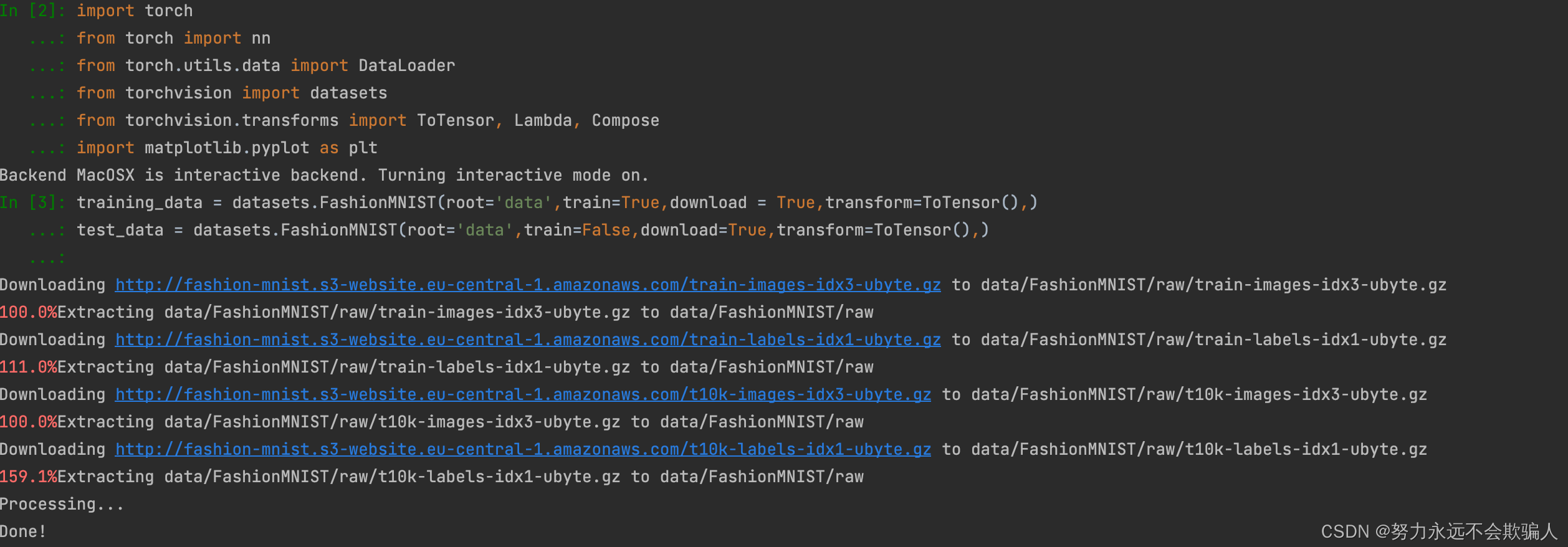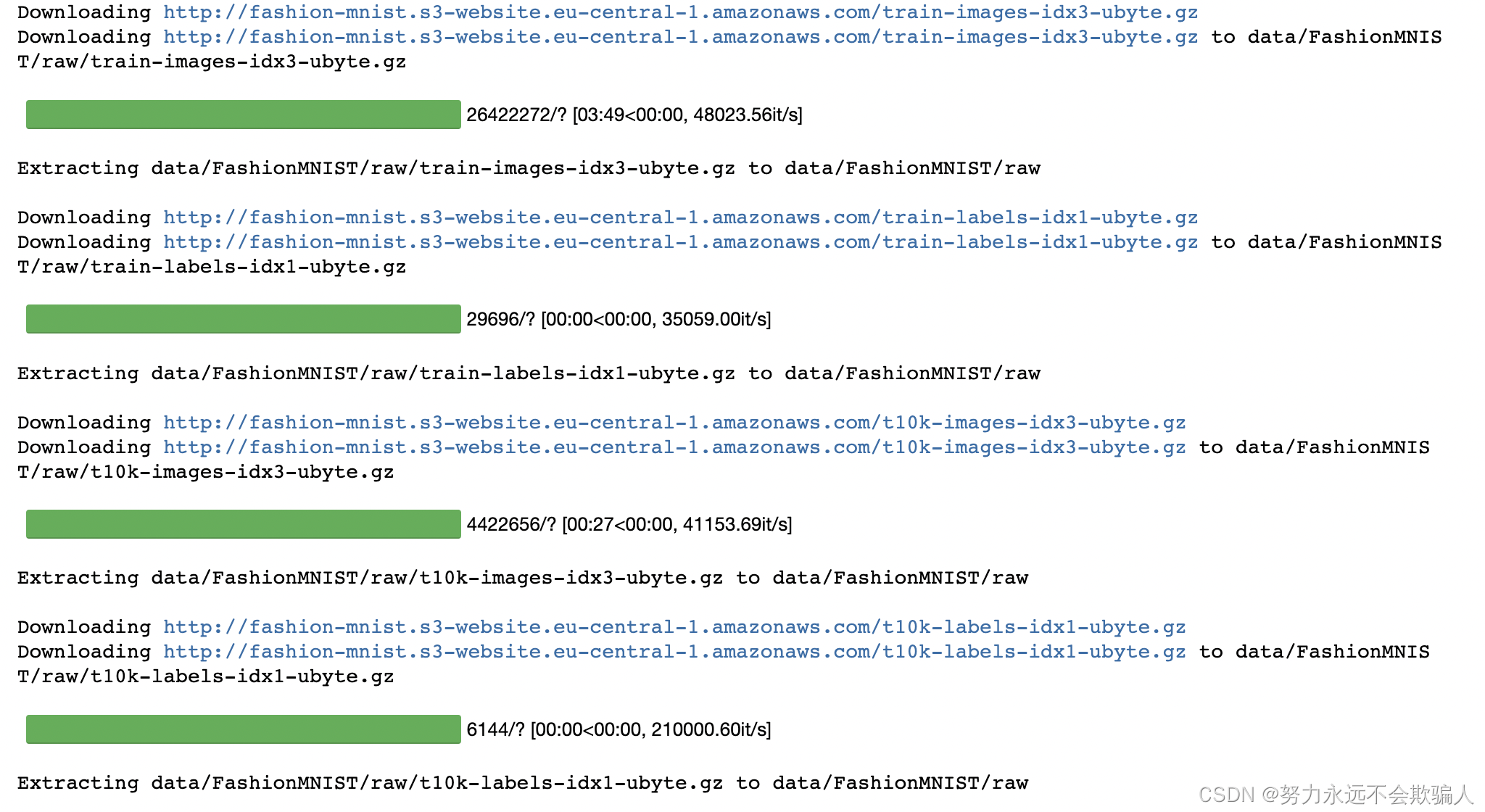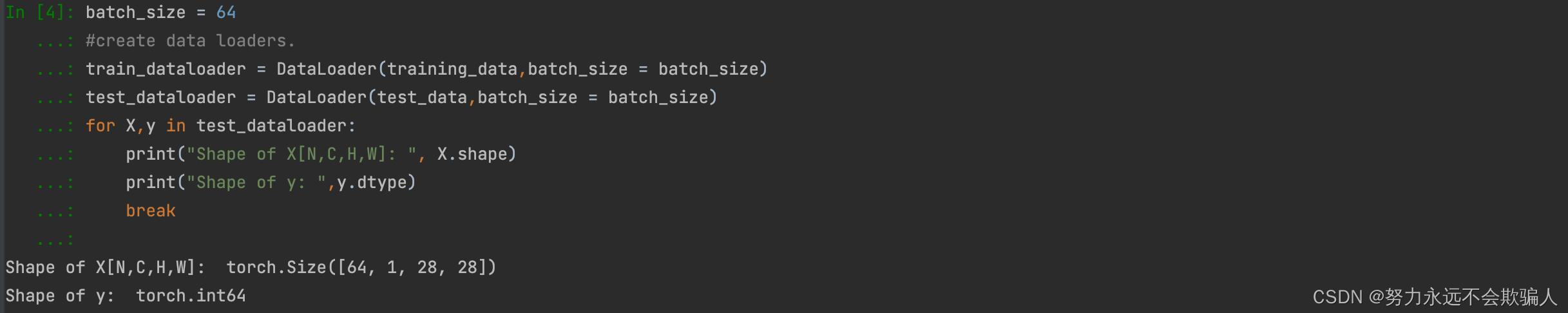### 1、2、2创建模型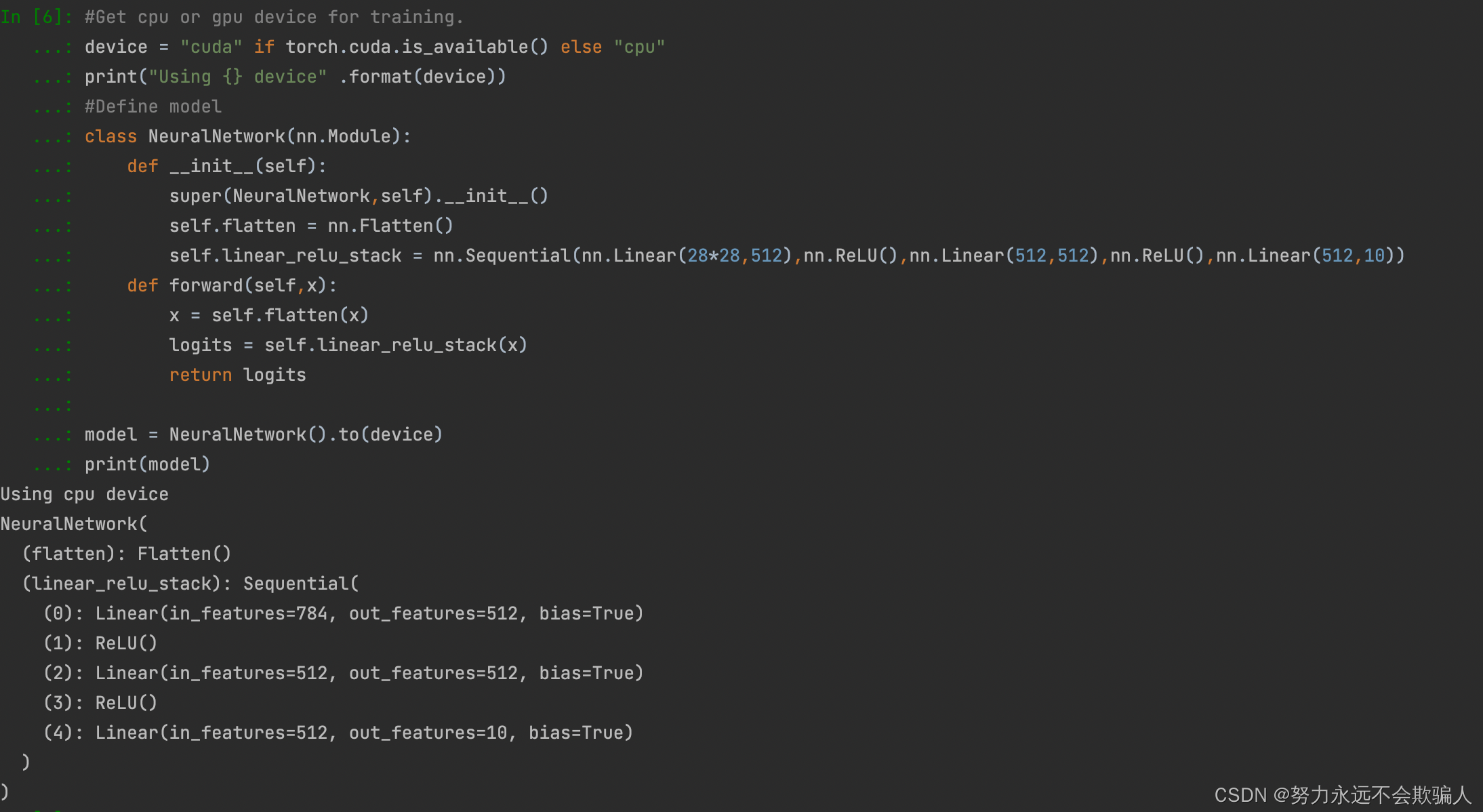flatten()函数用法

flatten是numpy.ndarray.flatten的一个函数，即返回一个一维数组

flatten只能适用于numpy对象，即array或者mat，普通的list列表不适用

### 1、2、3优化模型参数

``````loss_fn = nn.CrossEntropyLoss()
optimizer = torch.optim.SGD(model.parameters,lr = 1e-3)``````

``````def train(dataloader,model,loss_fn,optimizer):
model.train()
for batch, (X,y) in enumerate (dataloader):
X,y = X.to(device), y.to(device)

#Compute prediction error
pred = model(X)
loss = loss_fn(pred,y)

#Backpropagation
loss.backward()
optimizer.step()

if batch % 100 == 0:
loss,current = loss.item(), batch * len(X)
print(f"loss: {loss:>7f} [{current:>5d} / {size:>5d}]")``````

``````#根据测试数据集监测模型性能
model.eval()
test_loss,correct = 0,0
X,y = X.to(tensor), y.to(tensor)
pred = model(X)
test_loss += loss_fn(pred,y).item()
correct += (pred.argmax(1) == y).type(toech.float).sum().item()
test_loss /= num_batches
correct /= size
print(f"Test Error: \n Accuracy: {(100*correct):>0.1f}%,Avg loss:{test_loss:>8f} \n")``````

``````epochs = 5
for t in range(epochs):
print(f"Epoch {t+1}\n-------------------------")
print("Done!")``````

```Epoch 1
-------------------------
loss: 1.156493 [    0 / 60000]
loss: 1.171077 [ 6400 / 60000]
loss: 0.979696 [12800 / 60000]
loss: 1.117862 [19200 / 60000]
loss: 0.991124 [25600 / 60000]
loss: 1.021222 [32000 / 60000]
loss: 1.064837 [38400 / 60000]
loss: 1.001265 [44800 / 60000]
loss: 1.047198 [51200 / 60000]
loss: 0.965791 [57600 / 60000]
Test Error:
Accuracy: 65.7%,Avg loss:0.984534

Epoch 2
-------------------------
loss: 1.040607 [    0 / 60000]
loss: 1.074578 [ 6400 / 60000]
loss: 0.867450 [12800 / 60000]
loss: 1.026376 [19200 / 60000]
loss: 0.905349 [25600 / 60000]
loss: 0.927424 [32000 / 60000]
loss: 0.987350 [38400 / 60000]
loss: 0.926713 [44800 / 60000]
loss: 0.968327 [51200 / 60000]
loss: 0.898793 [57600 / 60000]
Test Error:
Accuracy: 66.7%,Avg loss:0.912300

Epoch 3
-------------------------
loss: 0.953451 [    0 / 60000]
loss: 1.006197 [ 6400 / 60000]
loss: 0.785461 [12800 / 60000]
loss: 0.960918 [19200 / 60000]
loss: 0.848042 [25600 / 60000]
loss: 0.857450 [32000 / 60000]
loss: 0.932683 [38400 / 60000]
loss: 0.875633 [44800 / 60000]
loss: 0.910384 [51200 / 60000]
loss: 0.850081 [57600 / 60000]
Test Error:
Accuracy: 67.9%,Avg loss:0.859877

Epoch 4
-------------------------
loss: 0.885792 [    0 / 60000]
loss: 0.954469 [ 6400 / 60000]
loss: 0.723592 [12800 / 60000]
loss: 0.911645 [19200 / 60000]
loss: 0.807442 [25600 / 60000]
loss: 0.804527 [32000 / 60000]
loss: 0.891161 [38400 / 60000]
loss: 0.839526 [44800 / 60000]
loss: 0.866708 [51200 / 60000]
loss: 0.812328 [57600 / 60000]
Test Error:
Accuracy: 69.1%,Avg loss:0.820029

Epoch 5
-------------------------
loss: 0.831598 [    0 / 60000]
loss: 0.913053 [ 6400 / 60000]
loss: 0.675240 [12800 / 60000]
loss: 0.873379 [19200 / 60000]
loss: 0.776931 [25600 / 60000]
loss: 0.763822 [32000 / 60000]
loss: 0.857460 [38400 / 60000]
loss: 0.812635 [44800 / 60000]
loss: 0.832454 [51200 / 60000]
loss: 0.781857 [57600 / 60000]
Test Error:
Accuracy: 70.6%,Avg loss:0.788298

Done!```

### 1、2、4保存模型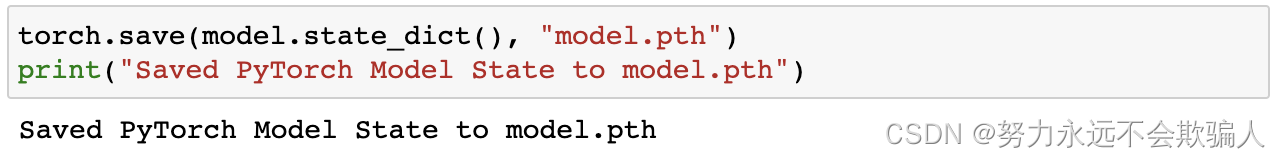### 1、2、5载入模型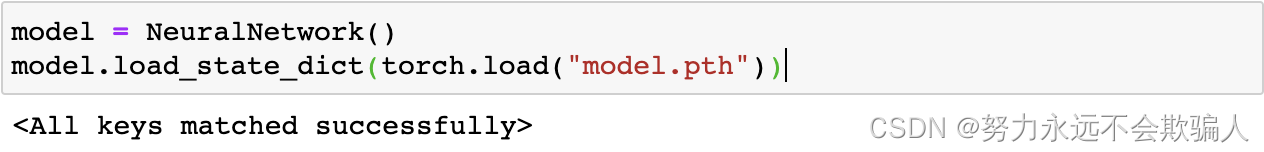这个模型现在可以用来进行预测

``````# 进行预测
classes = ["T-shirt/top","Trouser","Pullover","Dress","Coat","Sandal","Shirt","Sneaker","Bags","Ankle boot",]
model.eval()
x,y = test_data,test_data
pred = model(X)
predicted, actual = classes[pred.argmax(0)],classes[y]
print(f'Predicted: "{predicted}",Actual:"{actual}"')``````## 1、3 tensors

tensor是一种专有的数据结构，与数组和矩阵非常相似。在PyTorch中，我们使用tensor来编码一个模型的输入和输出，以及模型的参数。### 1、3、1 初始化tensor

tensor可以通过各种方式进行初始化。请看下面的例子。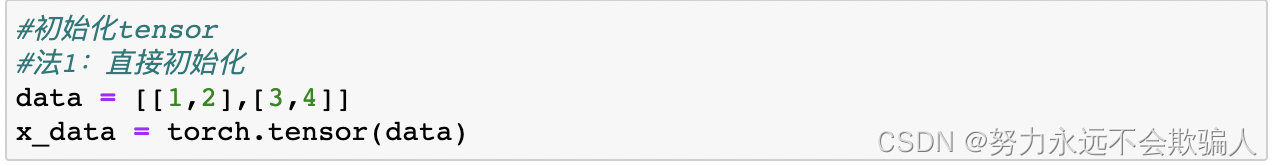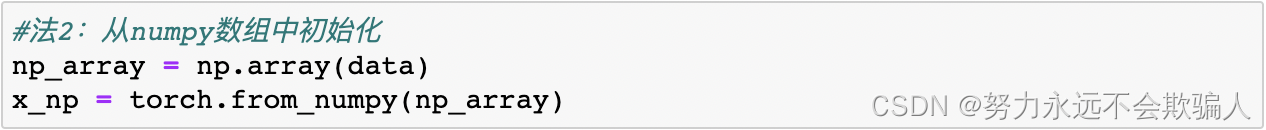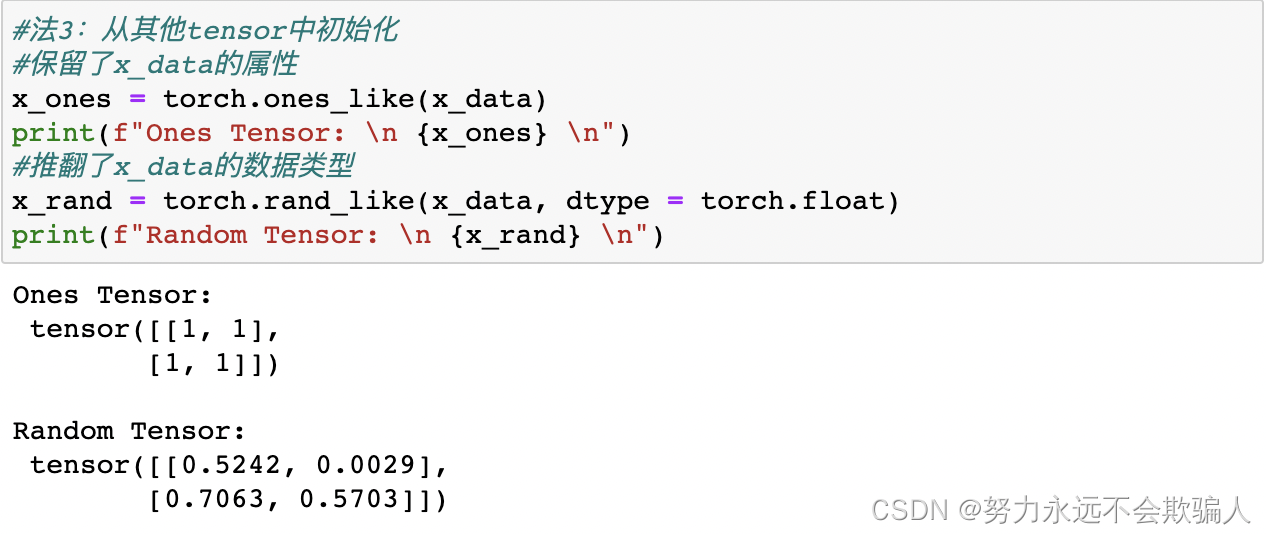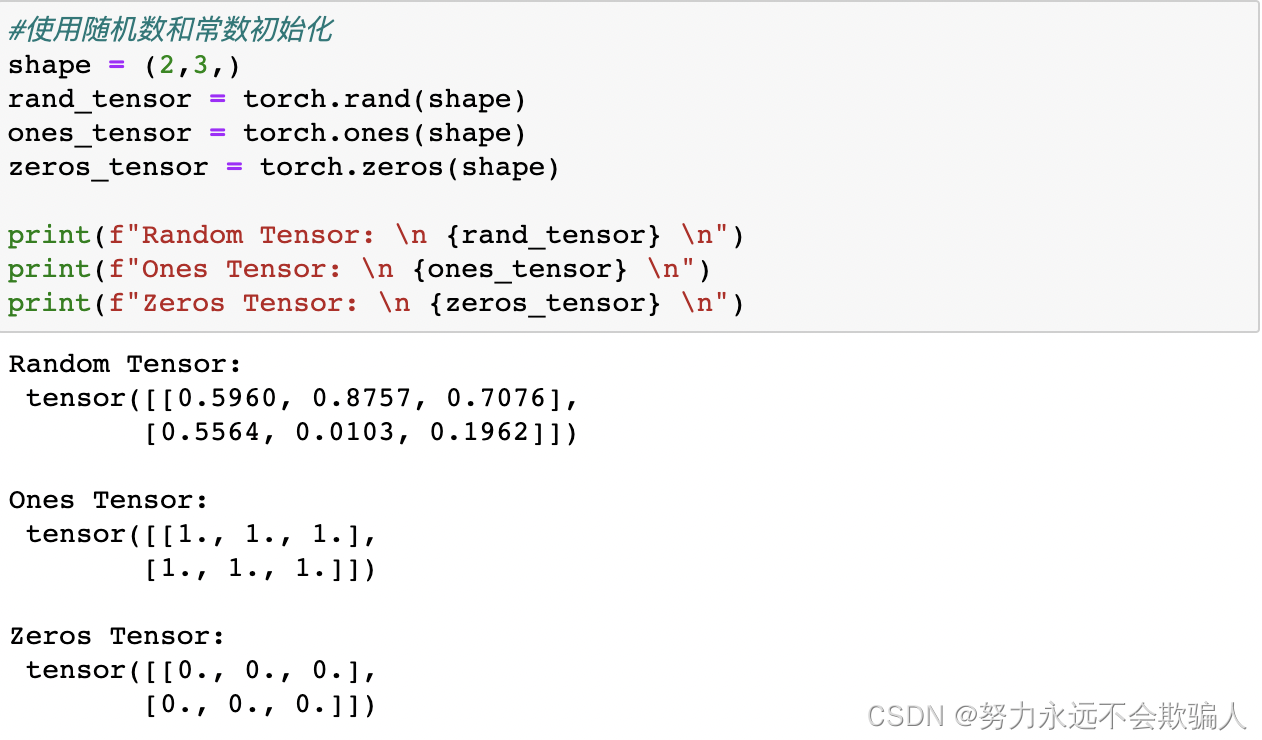### 1、3、2 tensor的性质

tensor属性描述了它们的形状、数据类型以及存储它们的设备。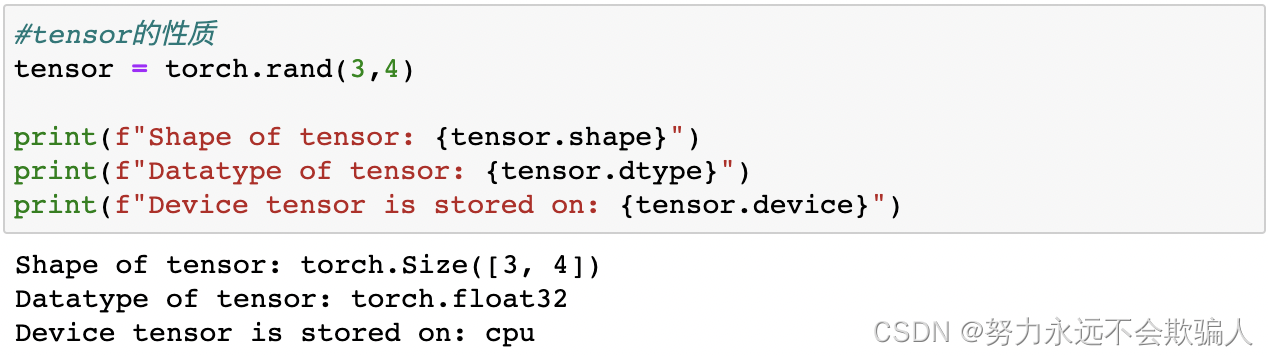### 1、3、3 tensor的操作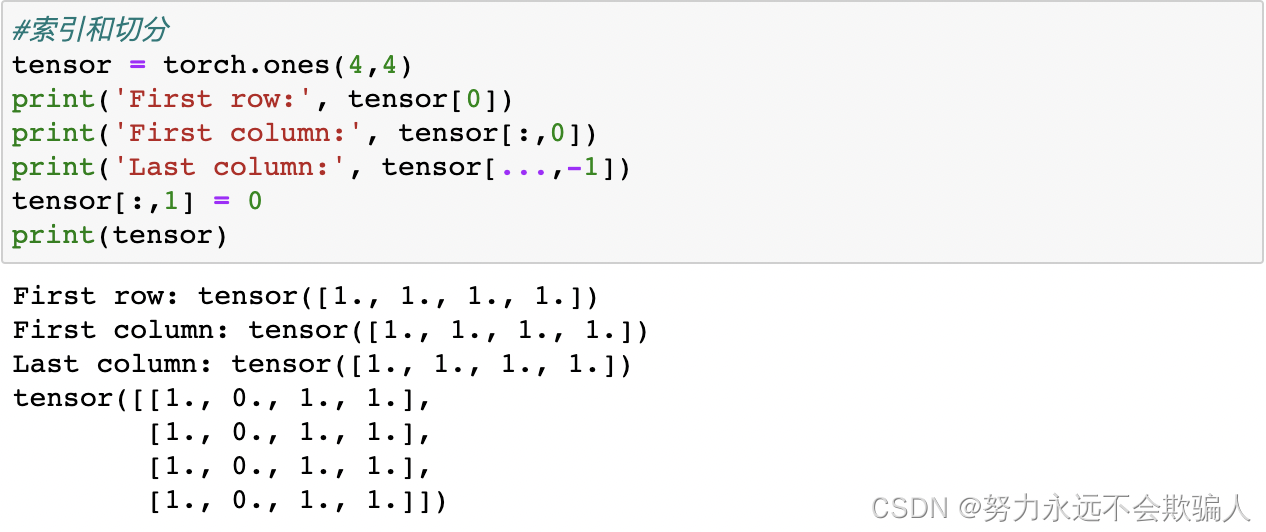b=a[;, ;, ;number]与b=a[;, ;, number]

[: , :, :number]，假设原来的a维度是（3X3X3），[: , :, :2]表示将原来的维度变为（3X3X2）。

[: , :, number]，假设原来的a维度是（3X3X3），[: , :, 2]表示将只保留标号为2的那一列。

**连接tensor:**你可以使用torch.cat沿着给定的维度连接多个tensor。也请看torch.stack，它是另一个与torch.cat有细微差别的tensor连接操作。

cvtutorials.com: torch.cat中的cat全称是concatnate,其中con是前缀，所以取cat代指concatenate，linux中的cat命令也是这个单词。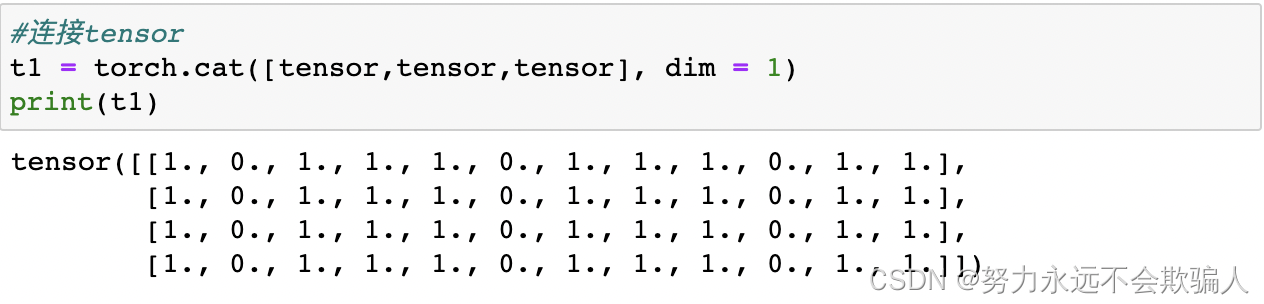dim = 1 在第一个维度进行拼接，即水平方向。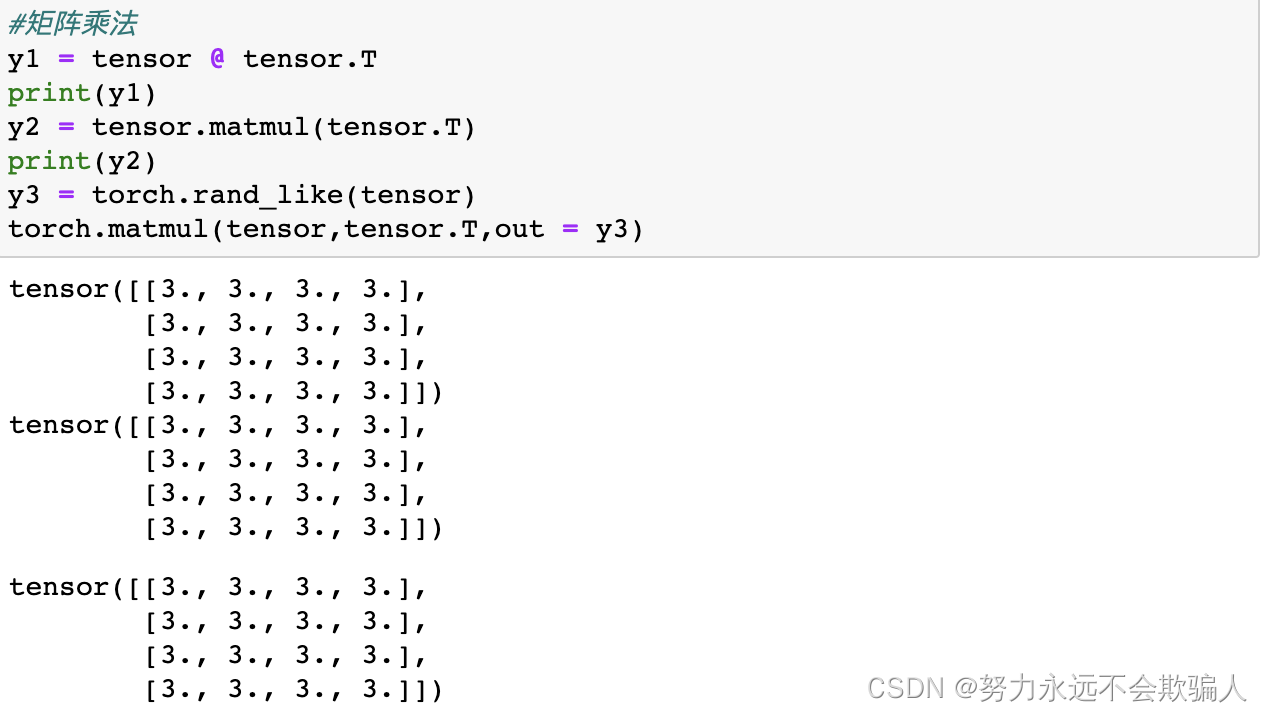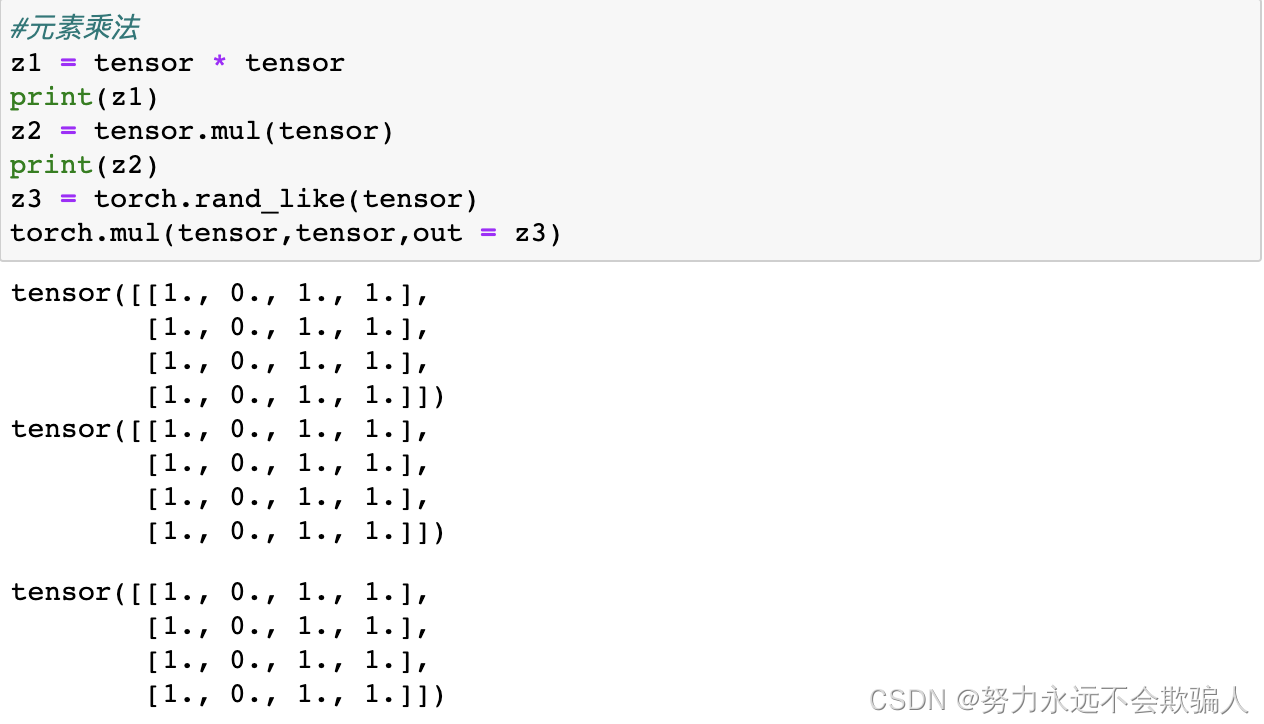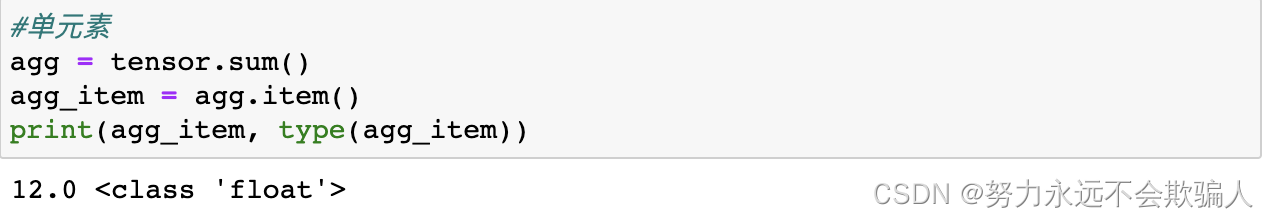原位操作 将结果存储到操作数中的操作被称为原位操作。它们用后缀来表示。例如：x.copy(y), x.t_(), 将改变x。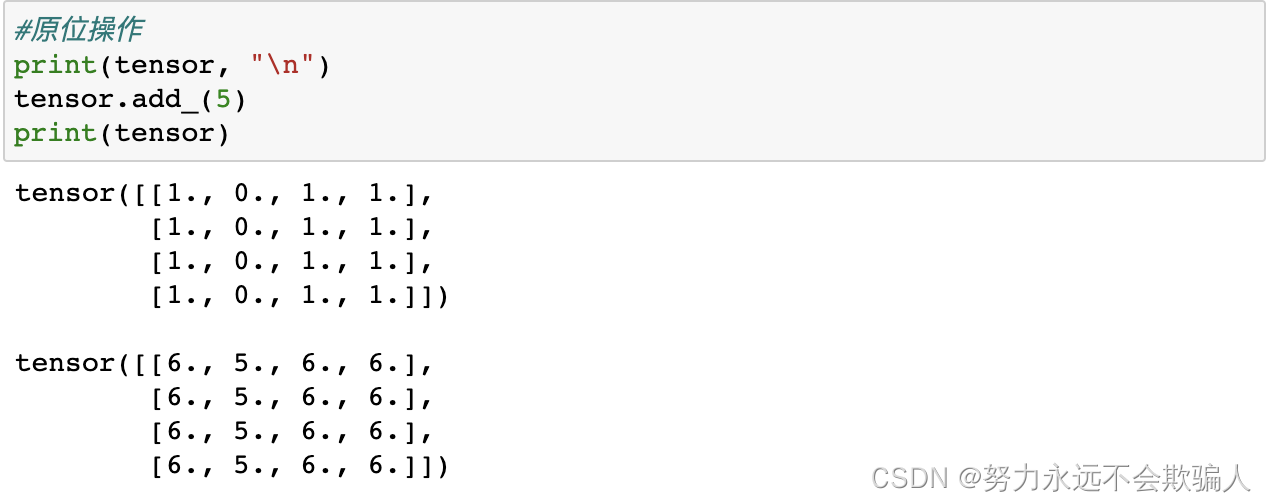### 1、3、4 和Numpy转换

CPU上的张量和NumPy数组可以共享它们的底层内存位置，改变一个将改变另一个

tensor转换为NumPy数组，tensor的变化反映在NumPy数组中。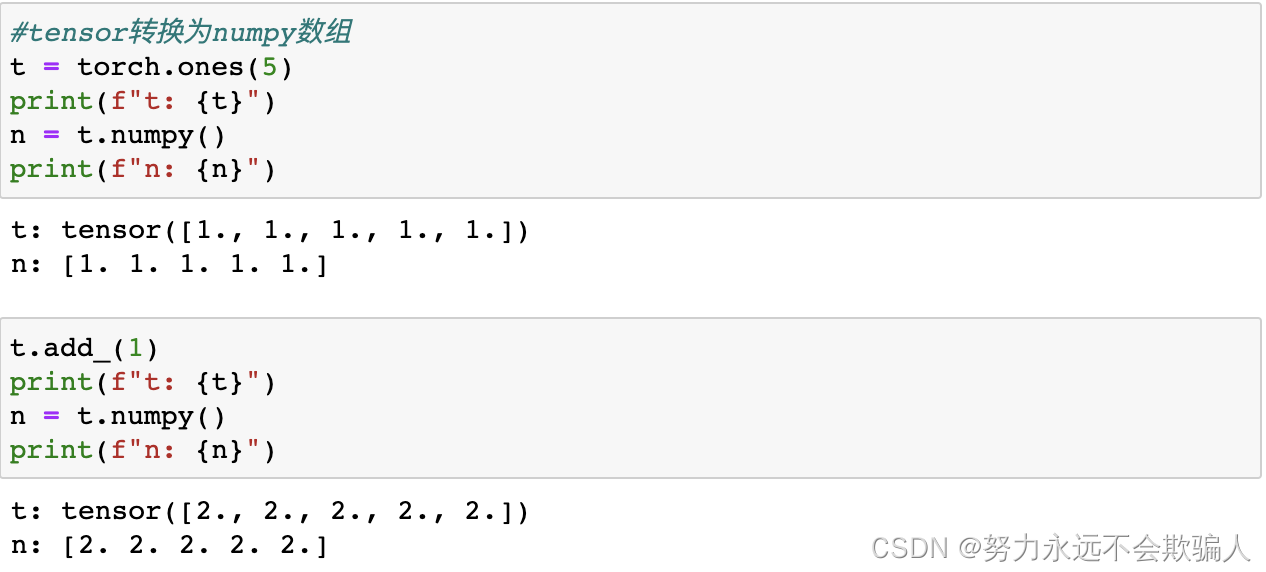Numpy数组转换为tensor，Numpy数组中的变化反映在tensor中。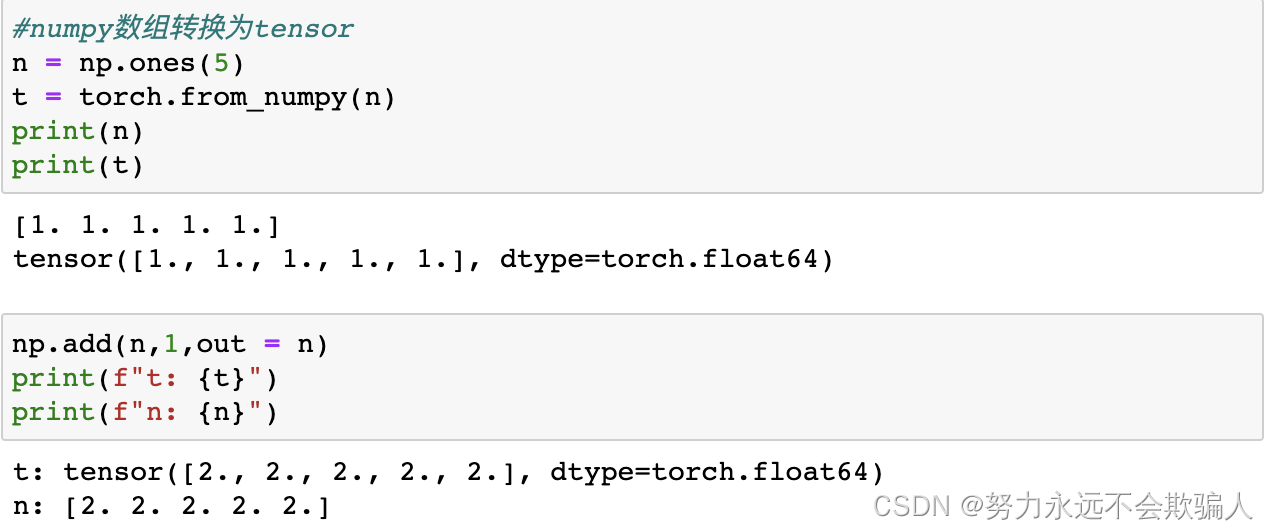## 1、4数据集和数据载入器

PyTorch库提供了一些预加载的数据集（如FashionMNIST），这些数据集是torch.utils.data.Dataset的子类，并实现了针对特定数据的功能。它们可以用来为你的模型建立原型和基准。

### 1、4、1 加载数据集

• root是存储训练/测试数据的路径
• train指定训练或测试数据集。
• download=True如果root目录下没有数据，则从互联网上下载数据，下载数据到`root`路径下。
• transform和target_transform指定特征和标签的变换

###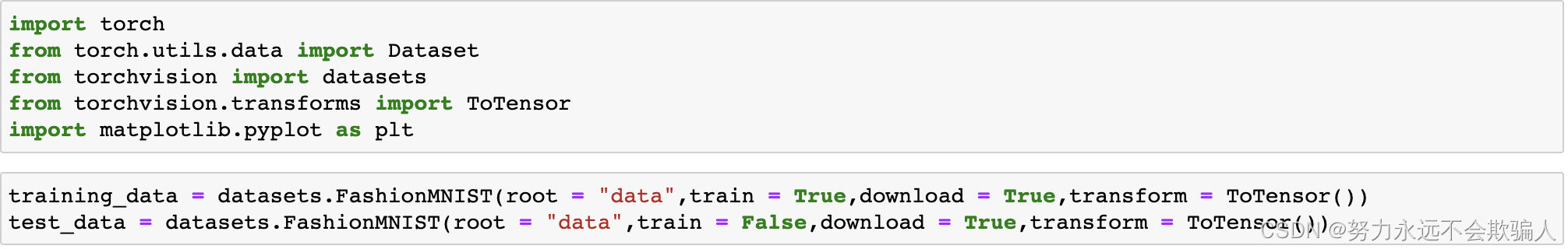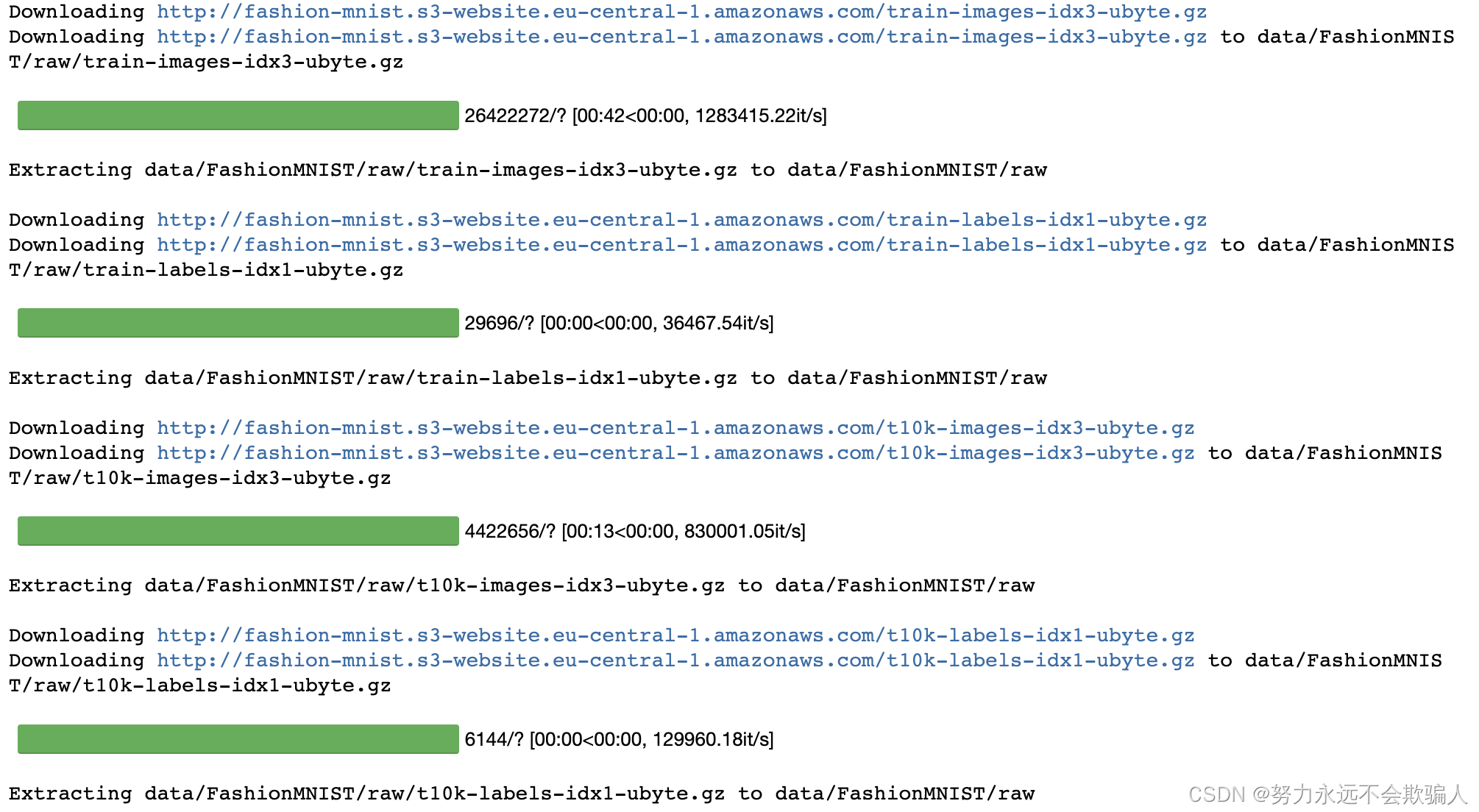1. root="data", # 数据集下载路径

2. train=True, # True为训练集，False为测试集

4. transform=ToTensor() # 对样本数据进行处理，转换为张量数据

### 1、4、2 数据集的迭代和可视化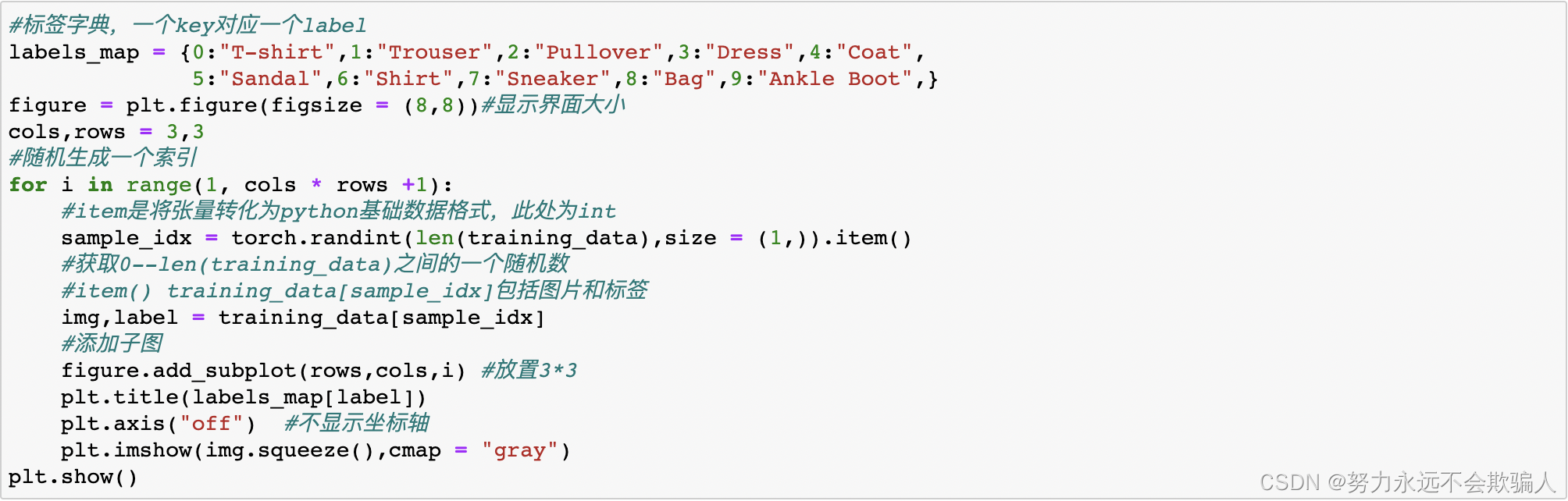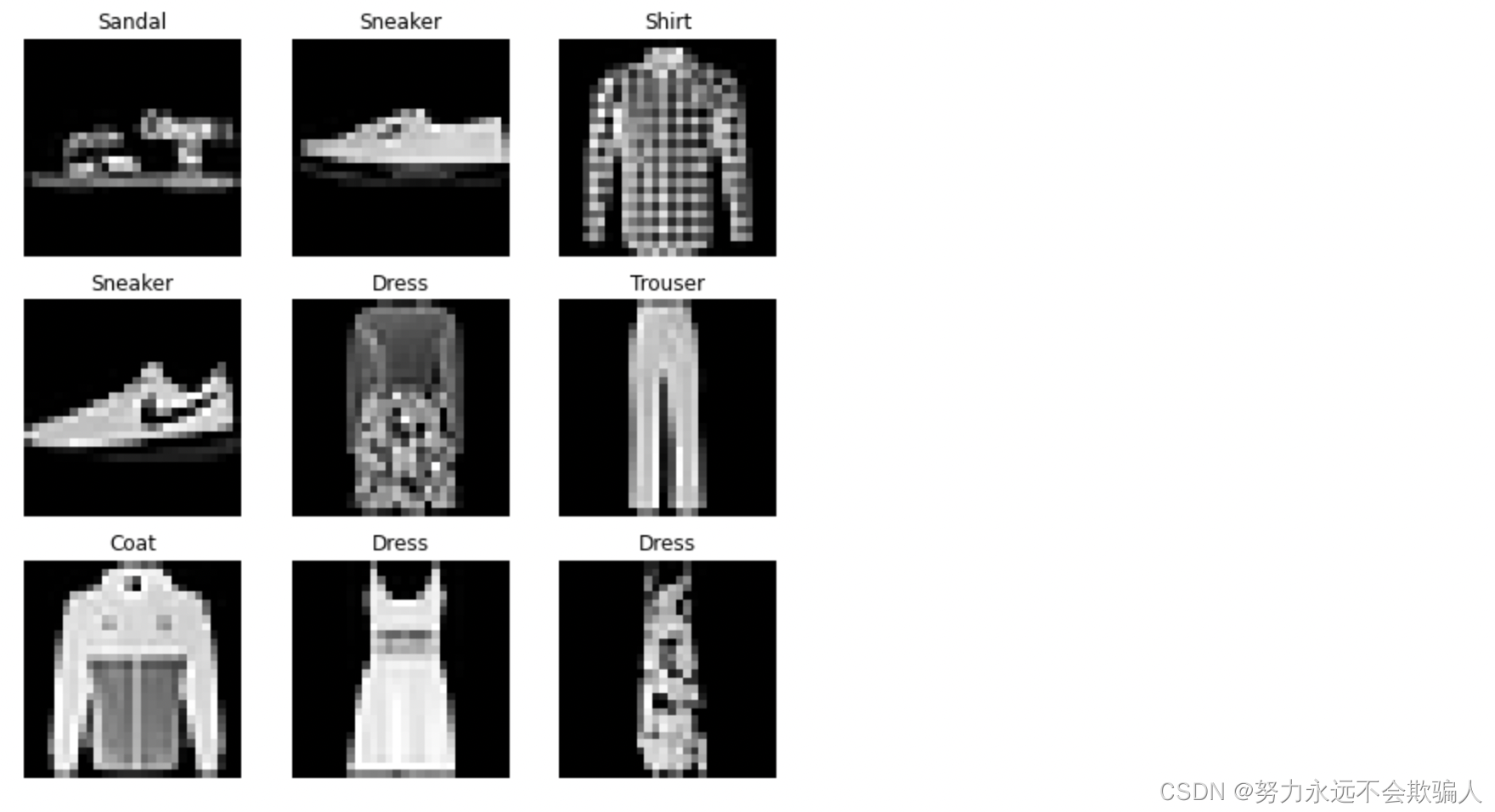### 1、4、3 为你的文件创建一个自定义数据集

• `__init__`：实例化Dataset对象时运行，完成初始化工作。初始化包含图像目录、标注文件目录和变换（在下一节有更详细的介绍）。
• `__len__`：返回数据集的大小。
• `__getitem__`：根据索引返回一个样本（数据和标签）。在给定的索引idx处加载并返回数据集中的一个样本。基于索引，它确定图像在磁盘上的位置，使用read_image将其变换为tensor，从self.img_labels中的csv数据中获取相应的标签，对其调用变换函数（如果适用），并在一个元组中返回tensor图像和相应标签。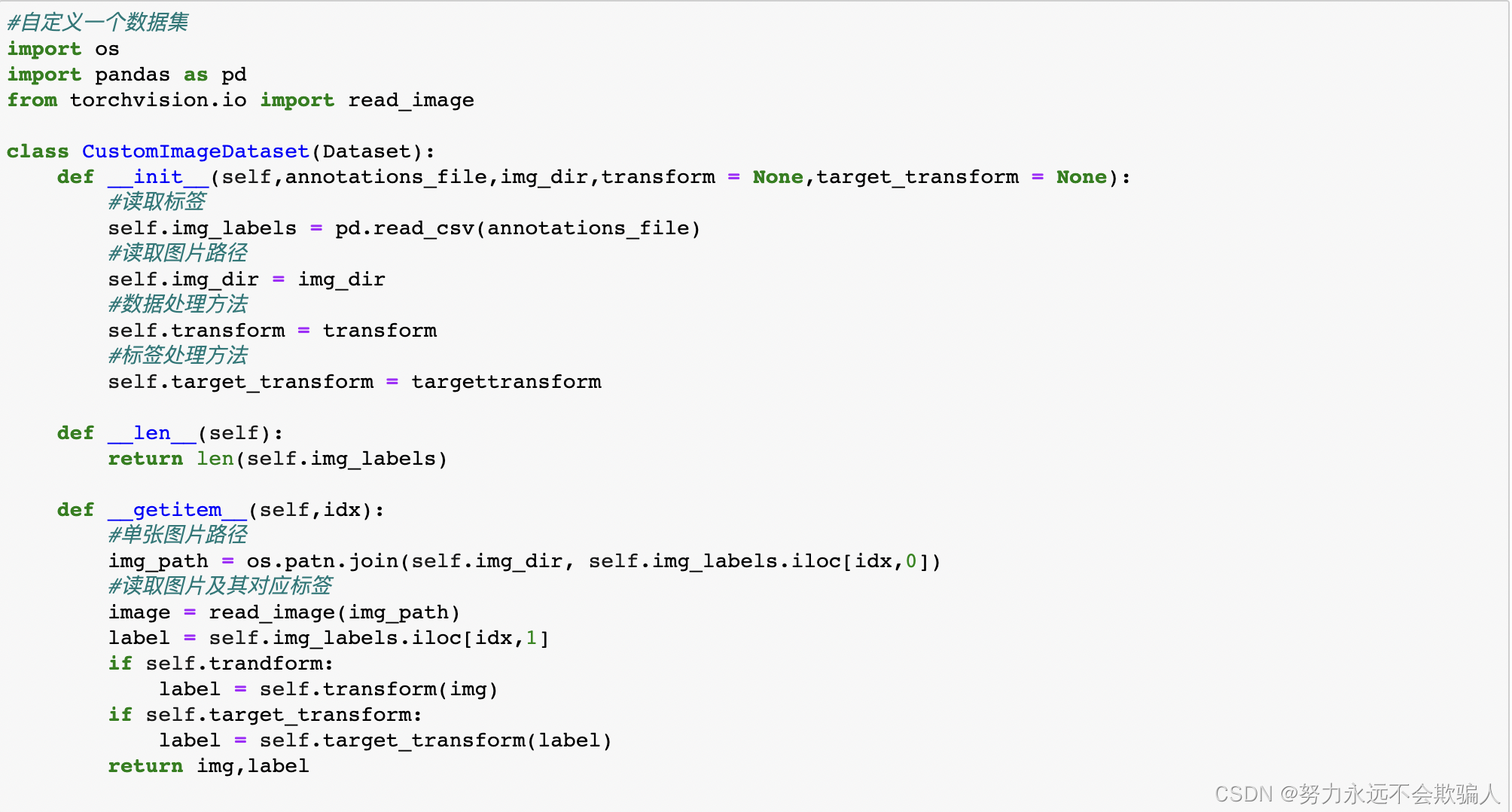• dataset (Dataset) ：定义好的数据集。
• batch_size (int, optional)：每次放入网络训练的批次大小，默认为1.
• shuffle (bool, optional) ：是否打乱数据的顺序，默认为False。一般训练集设置为True，测试集设置为False。
• num_workers (int, optional) ：线程数，默认为0。在Windows下设置大于0的数可能会报错。
• drop_last (bool, optional) ：是否丢弃最后一个批次的数据，默认为False。

• enumerate(iterable, start=0)：输入是一个可迭代的对象和下标索引开始值；返回可迭代对象的下标索引和数据本身。
• tqdm(iterable)：进度条可视化工具包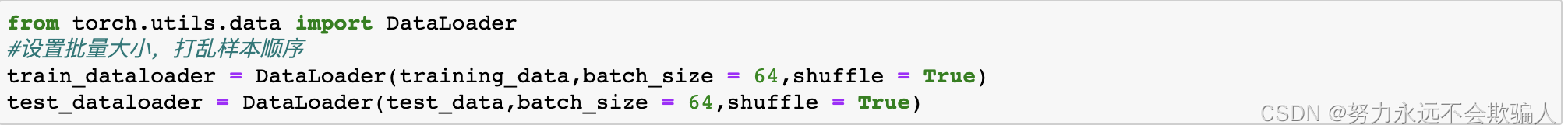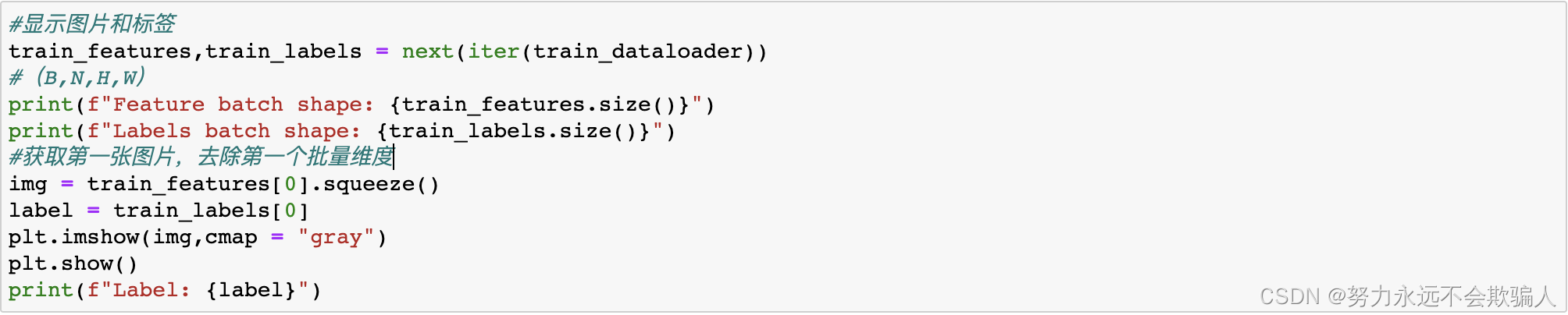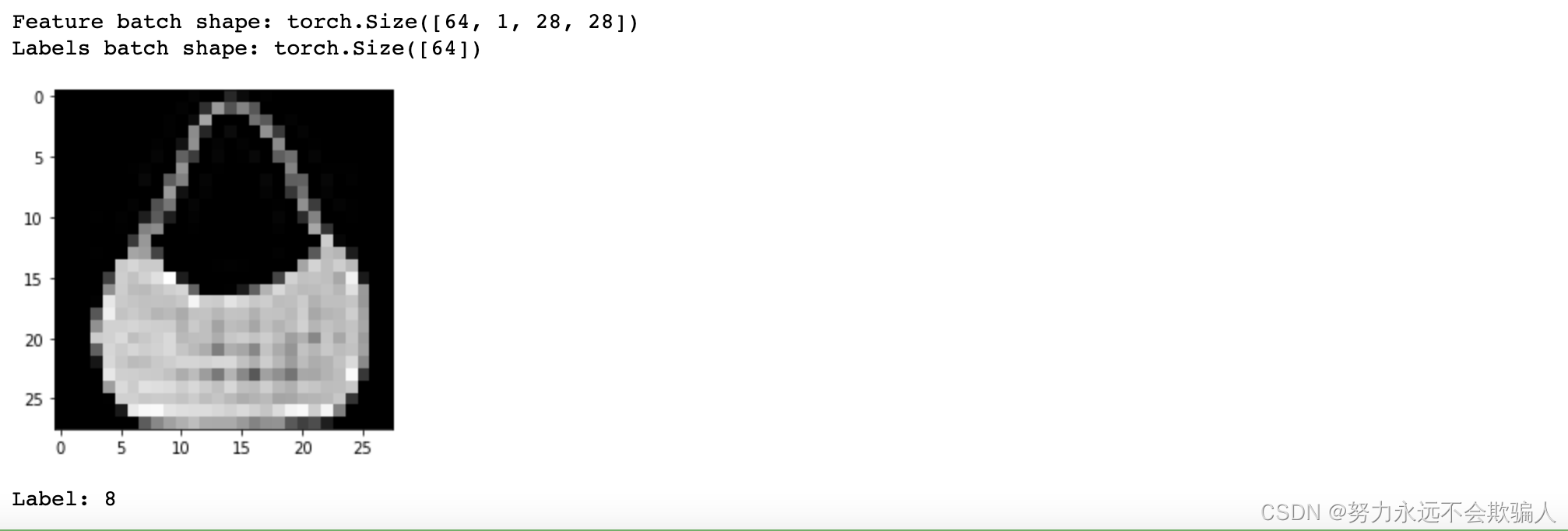## 1、5 变换

FashionMNIST的特征是PIL图像格式的，标签是整数。对于训练，我们需要将特征作为归一化的tensor，将标签作为one-hot编码的tensor。为了进行这些变换，我们使用ToTensor和Lambda。

Lambda变换

Lambda变换应用任何用户定义的Lambda函数。在这里，我们定义了一个函数，把整数变成一个one-hot的tensor。它首先创建一个大小为10（我们数据集中的标签数量），值为0的tensor，并调用scatter_，在标签y给出的索引上分配一个value=1。

transforms.ToTensor()

transforms.Normalize()

``output[channel] = (input[channel] - mean[channel]) / std[channel]``

transforms.ToPILImage()

transforms.Resize()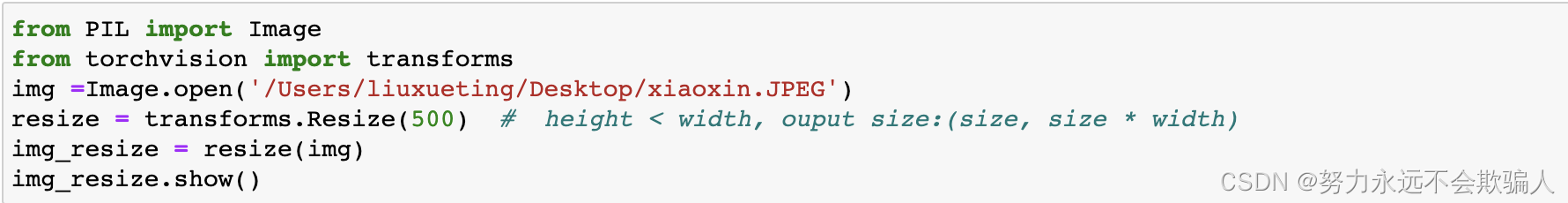原始图像大小为1280*2796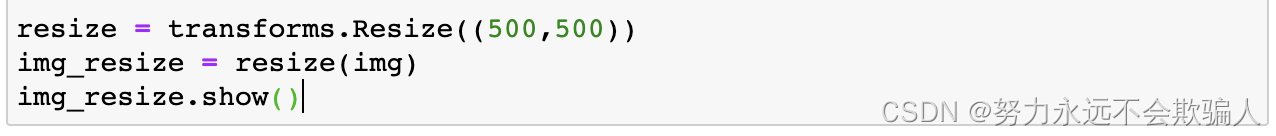transforms.CenterCrop()transforms.RandomCrop()transforms.RandomResizedCrop()

transforms.RandomHorizontalFlip()

transforms.RandomVerticalFlip()

transforms.RandomRotation()

## 1、6 搭建神经网络

###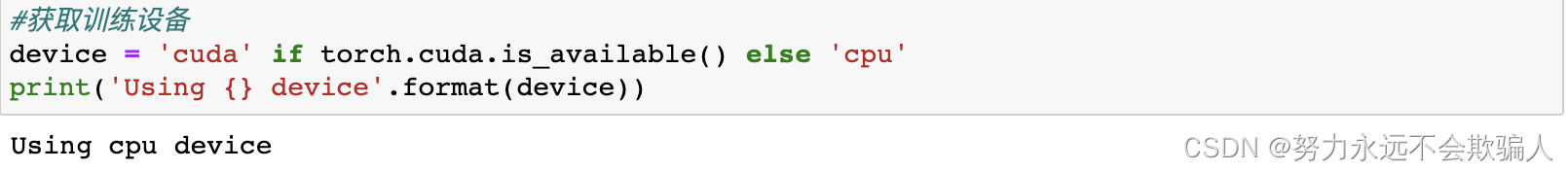1、6、2 定义类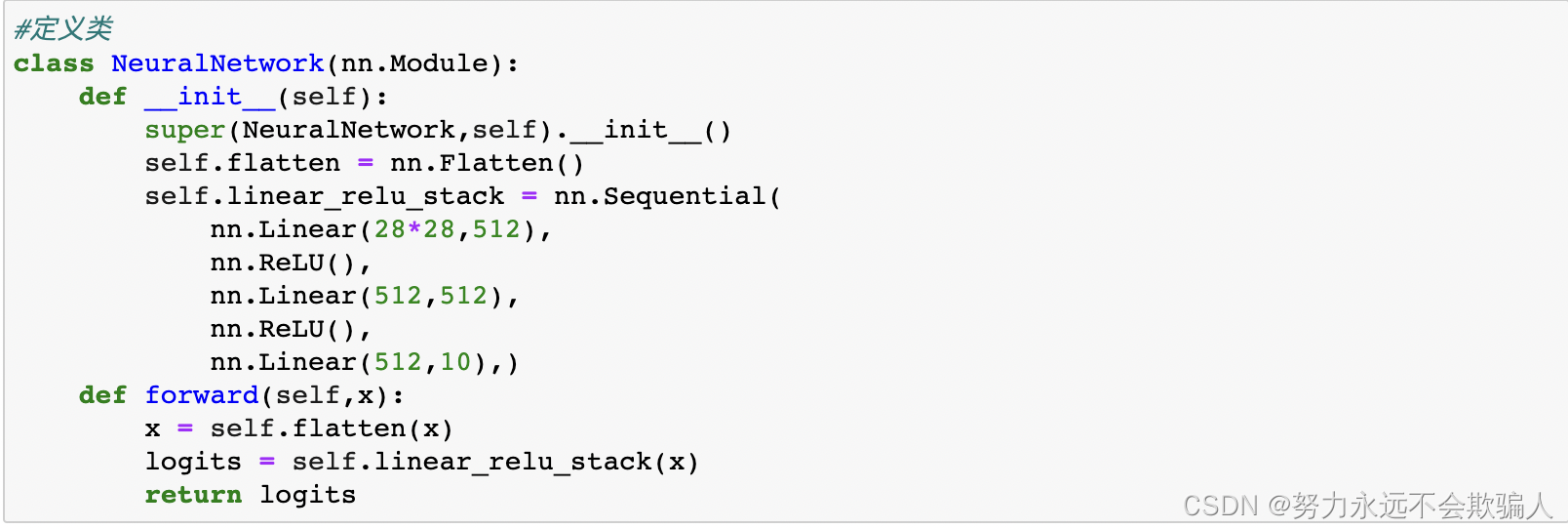实例化`NeuralNetwork`类，并将其移动到`device`上。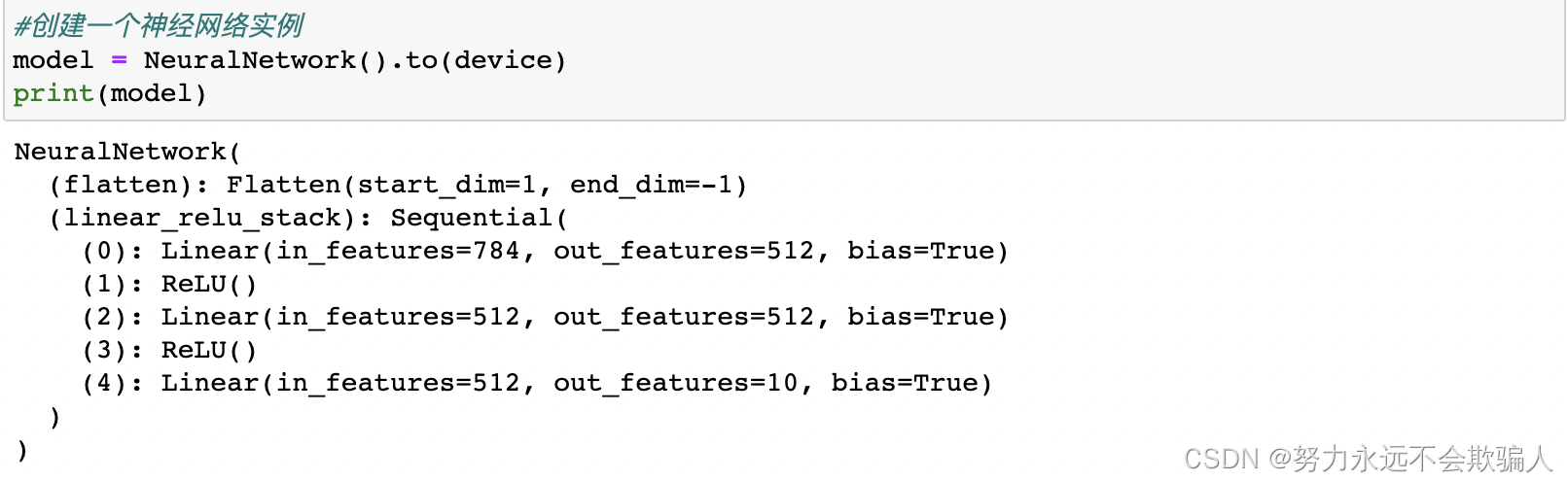为了使用这个模型，我们把输入数据传给它。这就执行了模型的forward函数，以及一些后台操作。请不要直接调用model.forward()!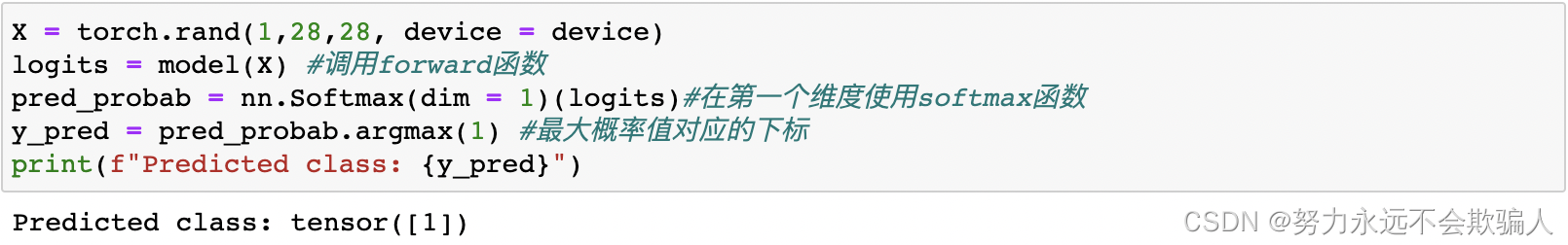### 1、6、3 模型层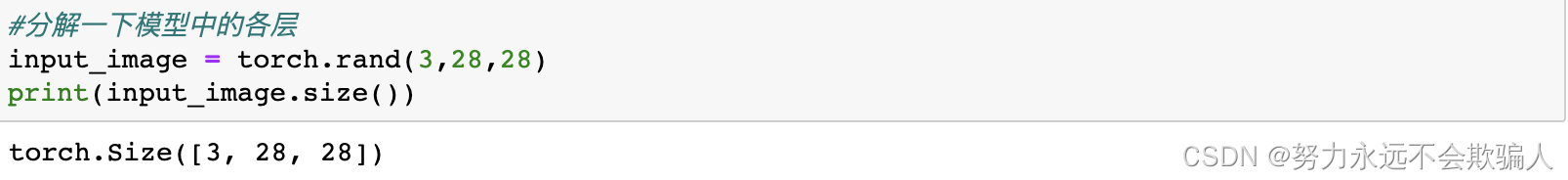nn.Flatten

`nn.Flatten`层以将每个大小为28x28的图像转换为784个像素值的连续数组（保持批量维度（dim=0））。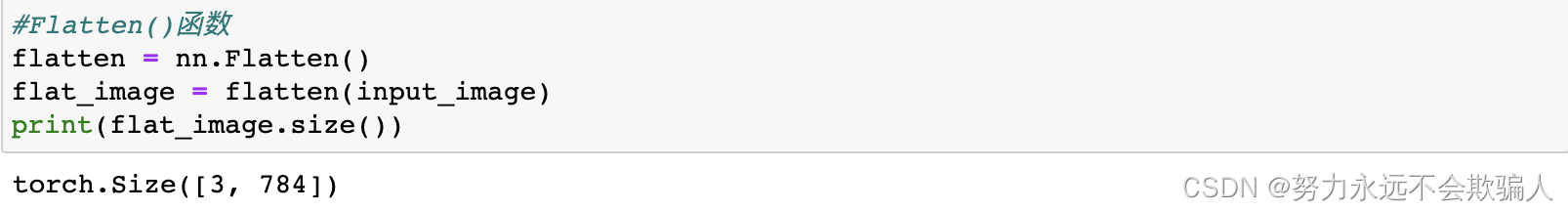nn.Linear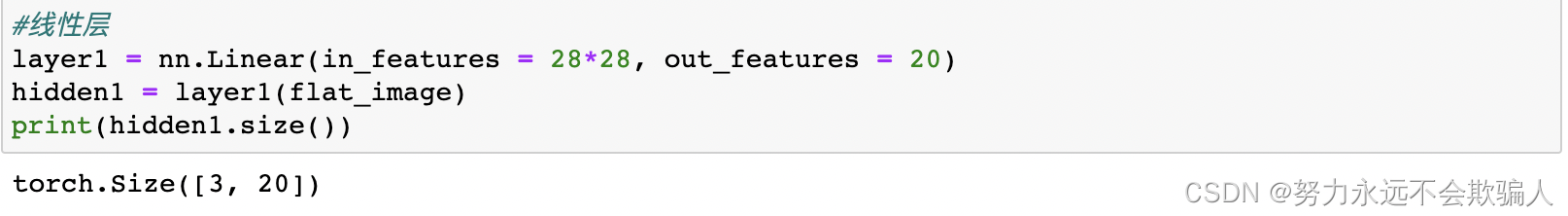nn.ReLU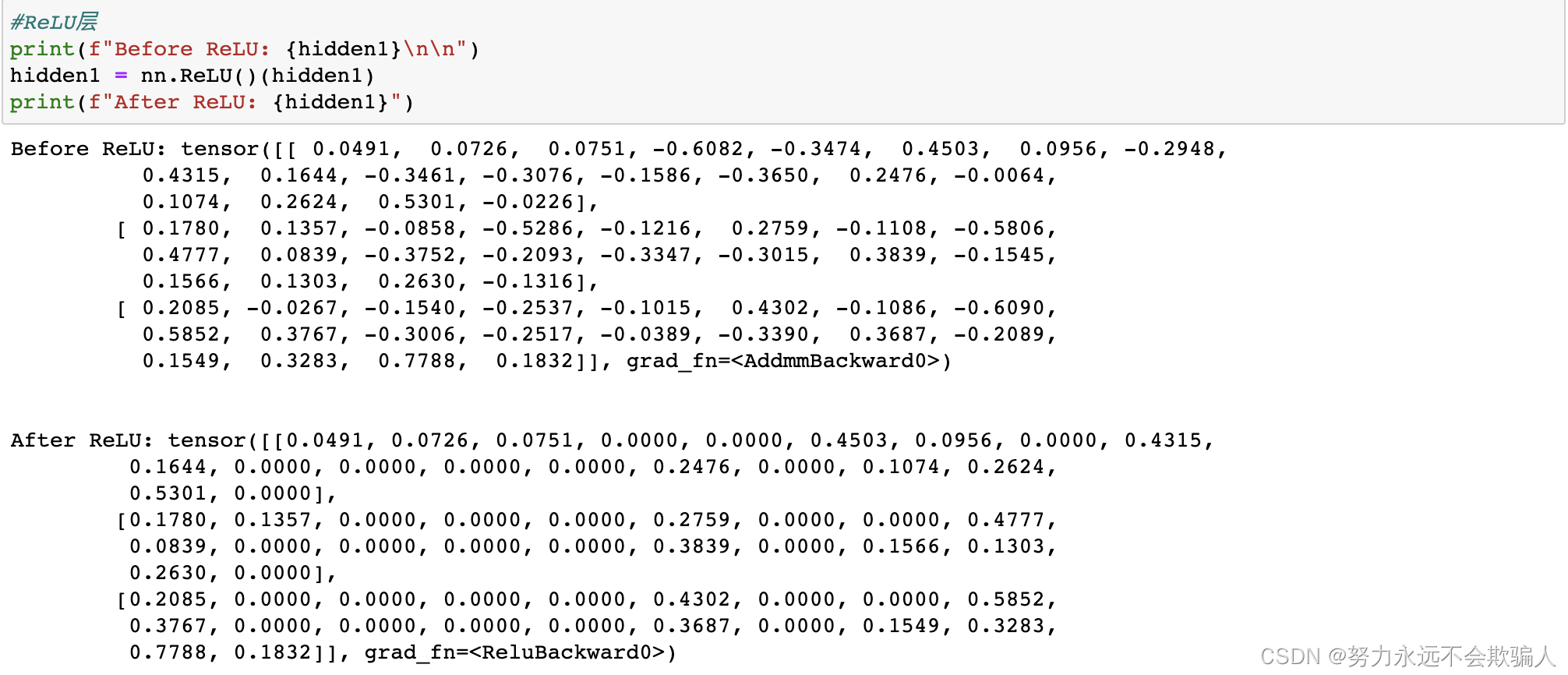引入非线性激活函数的原因：

nn.Sequential

`nn.Sequential`可以理解为网络层的容器，在其中我们定义各种网络层，数据会按照我们设置的顺序经过所有网络层。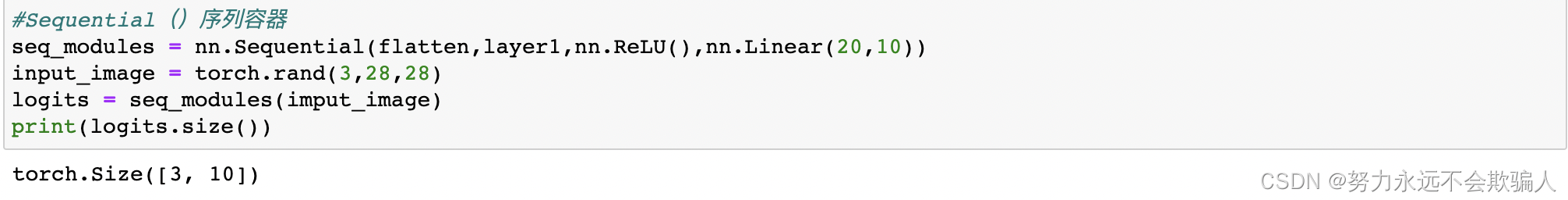nn.Softmax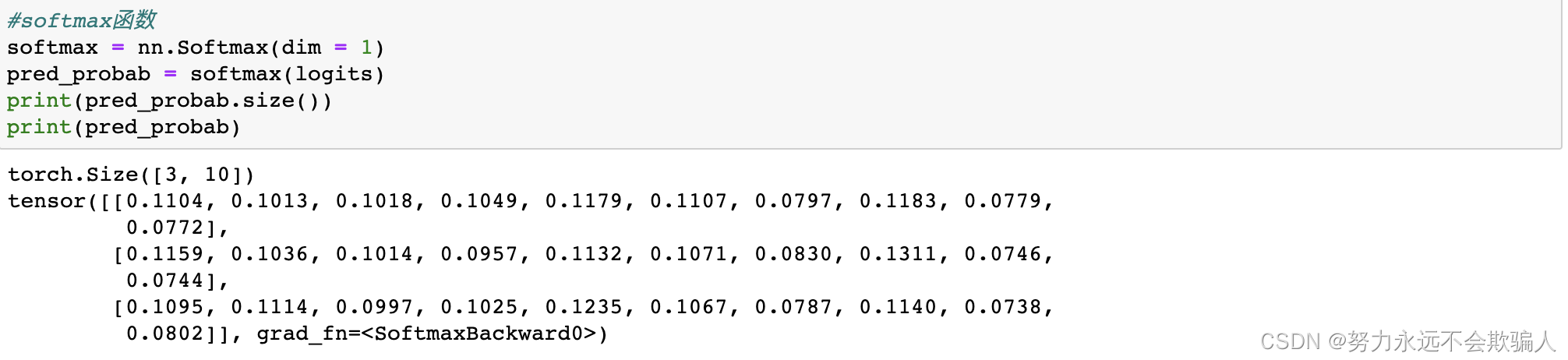### 1、6、4 模型参数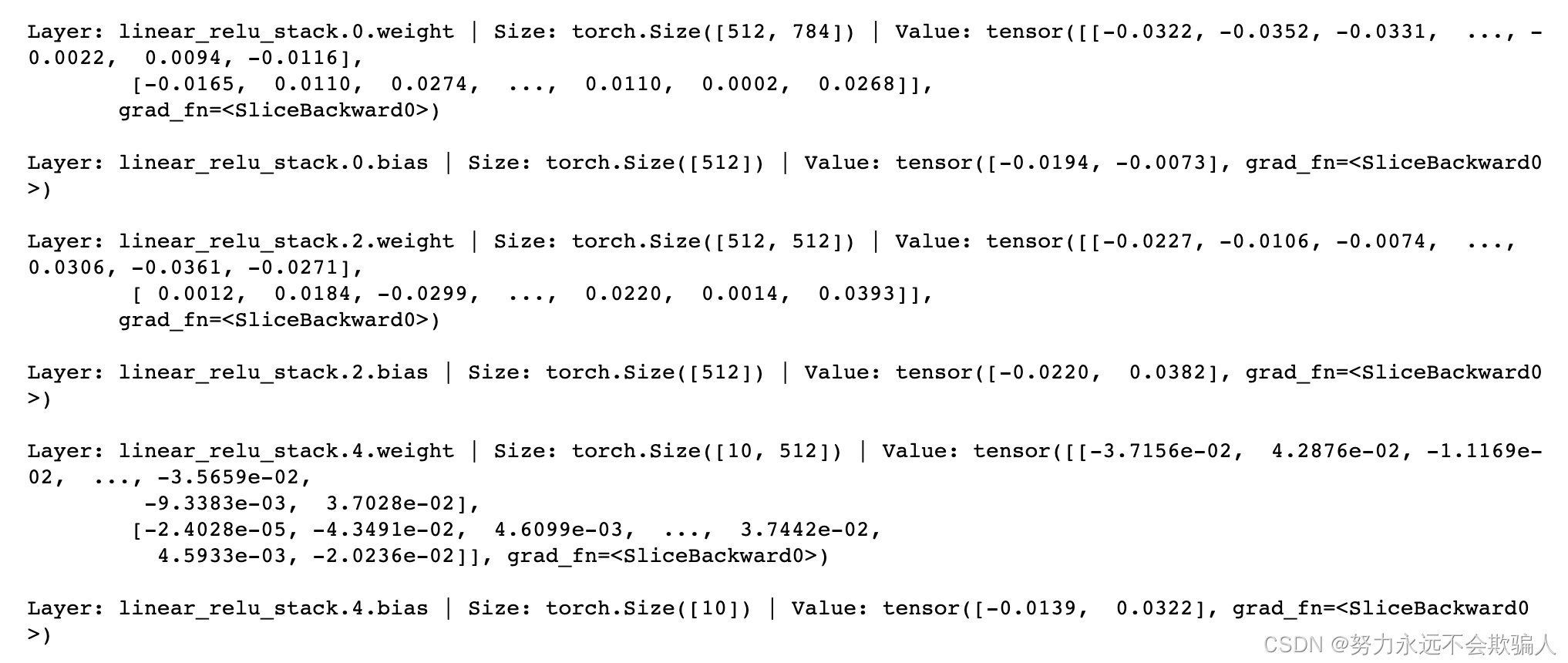###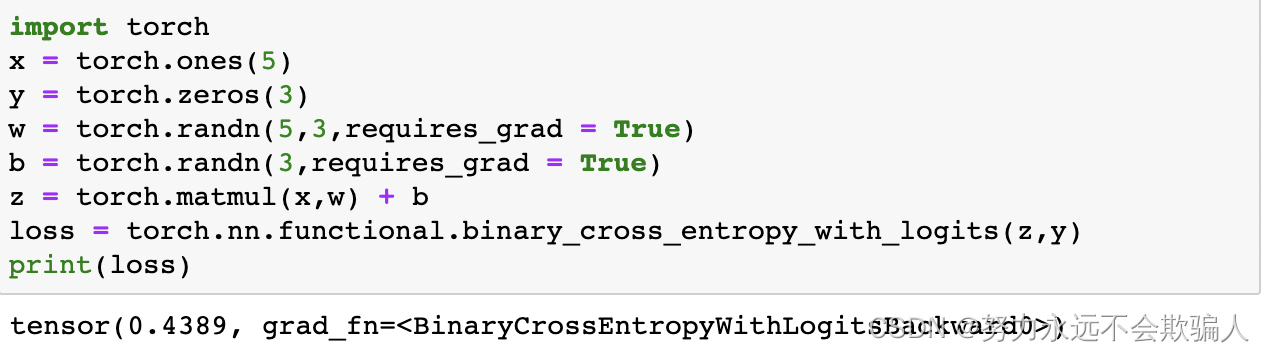1、7、1 tensor、函数和计算图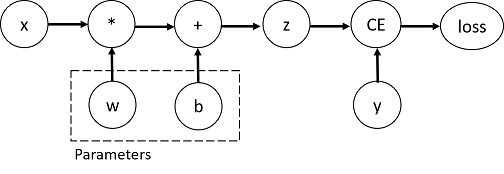### 构建计算图的函数是Function类的一个对象。这个对象知道如何计算正向的函数*，*以及如何在反向传播步骤中计算导数，可以通过张量的grad_fn属性查看。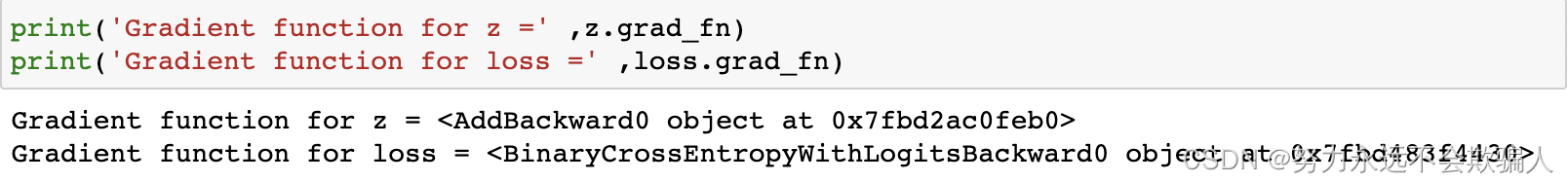1、7、2 计算梯度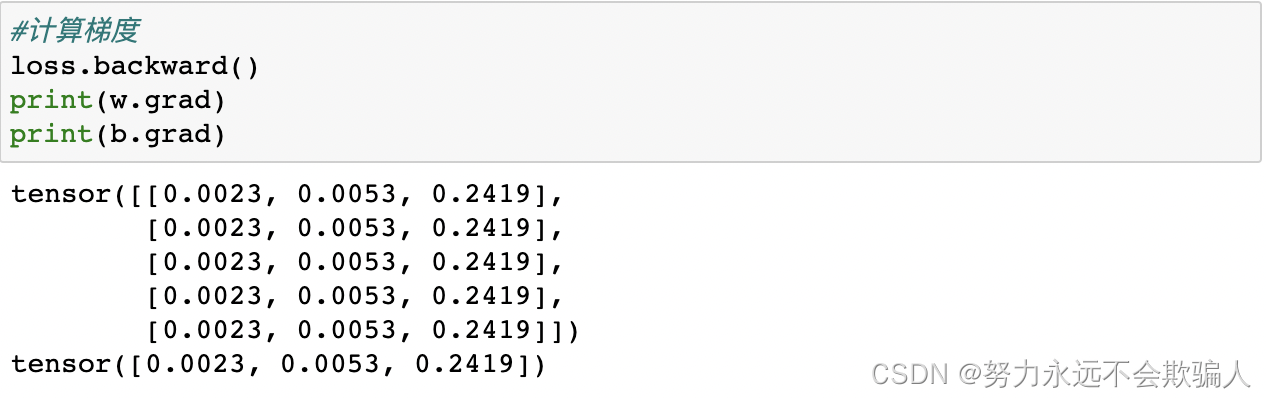• 出于性能方面的考虑，我们只能在一个给定的图上使用一次backward来进行梯度计算。如果我们需要在同一个图上进行多次backward调用，我们需要在backward调用中传递 retain_graph=True。

### 1、7、3 禁止梯度跟踪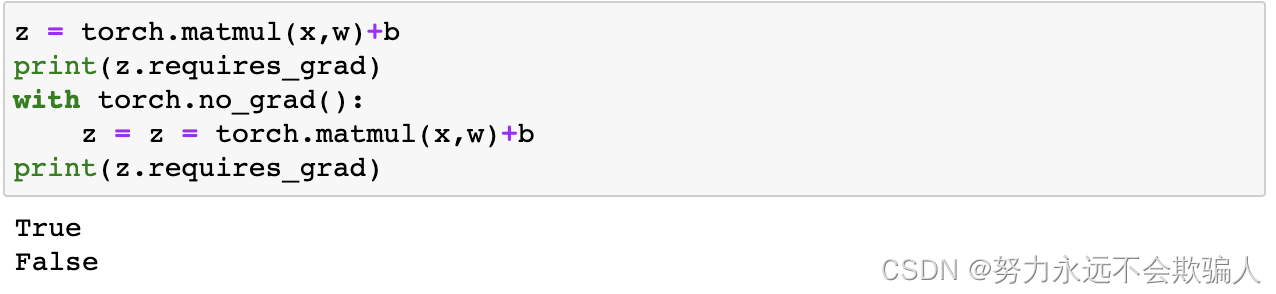另一种实现相同结果的方法是在tensor上使用detach()方法。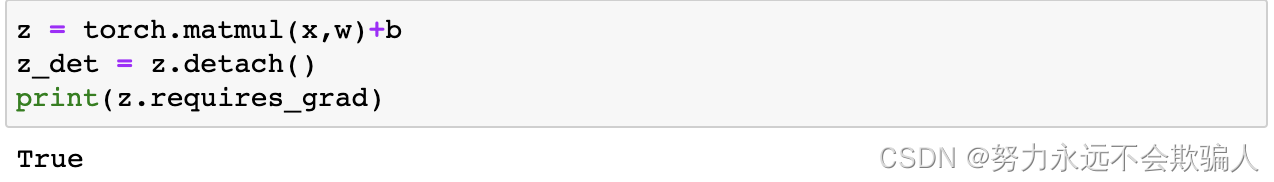你可能想禁用梯度跟踪，原因如下：

• 将神经网络中的一些参数标记为冻结参数。这是对预训练的网络进行微调的一个非常常见的情况。
• 当你只做前向传递时，为了加快计算速度，对不跟踪梯度的tensor的计算会更有效率。

### 1、7、4 更多关于计算图

• 运行请求的操作，计算出一个结果tensor。
• 在DAG中维护该操作的梯度函数。

• 使用链式规则，一直传播到叶子tensor。

PyTorch允许你计算雅各布乘积，而不是计算雅各布矩阵本身。对于一个给定的输入矢量v=(v_1...v_m)。 这可以通过调用v作为参数的backward来实现。v的大小应该与原始张量的大小相同，我们要进行乘积计算。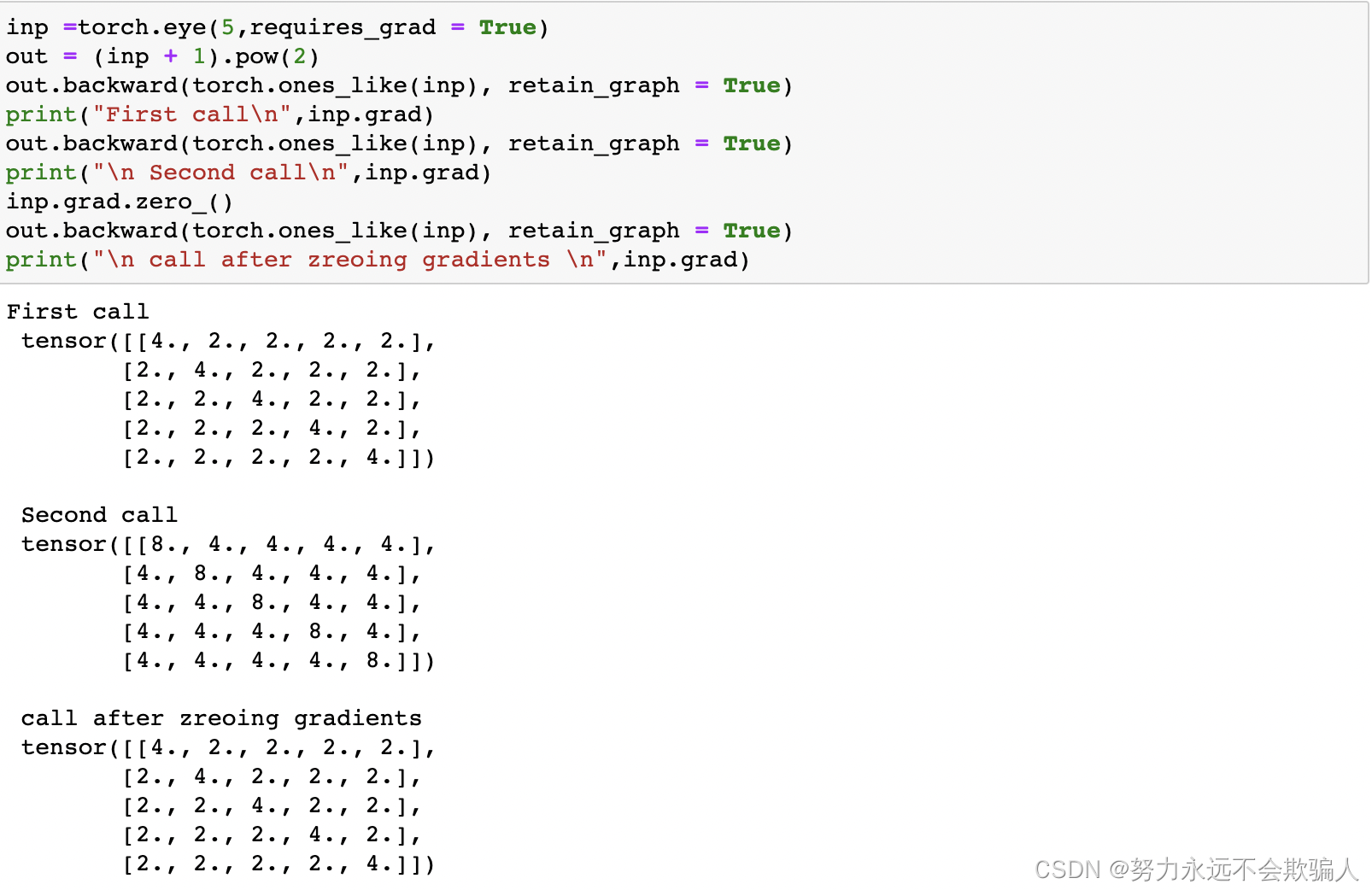## 1、8 优化模型参数

### 1、8、1 先决条件代码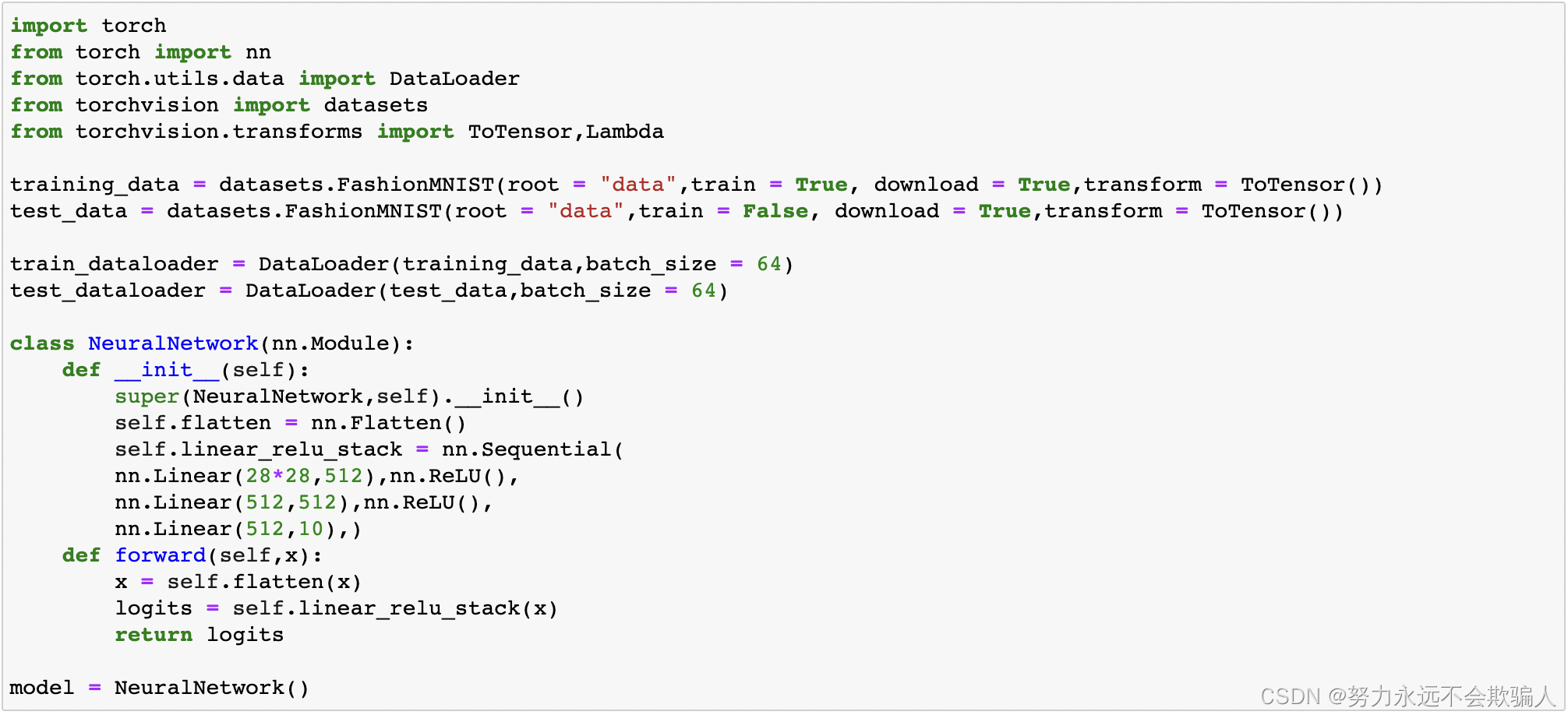### 1、8、2 超参数

• epoch数 - 在数据集上迭代的次数
• 批量大小--在更新参数之前，通过网络传播的数据样本的数量。
• 学习率--在每个批次/epoch更新模型参数的程度。较小的值产生缓慢的学习速度，而较大的值可能会导致训练期间的不可预测的行为。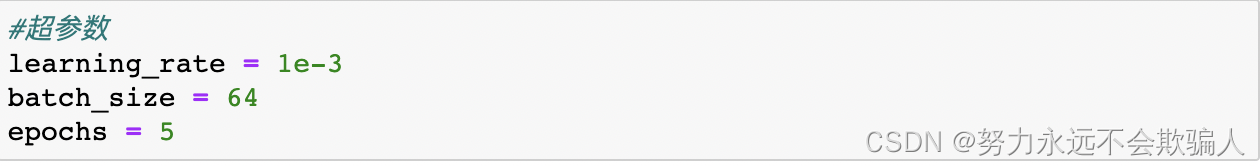### 1、8、3 优化Loop

• 训练loop--在训练数据集上迭代，试图收敛到最佳参数。
• 验证/测试循环--迭代测试数据集，以检查模型性能是否在提高。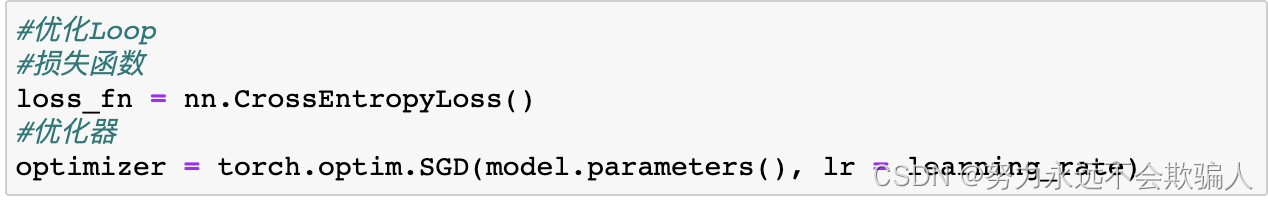• 通过调用loss.backwards()对预测损失进行反向传播。PyTorch将损失的梯度与每个参数联系在一起。
• 一旦我们有了梯度，我们就可以调用optimizer.step()来根据向后传递中收集的梯度调整参数。

### 1、8、4 执行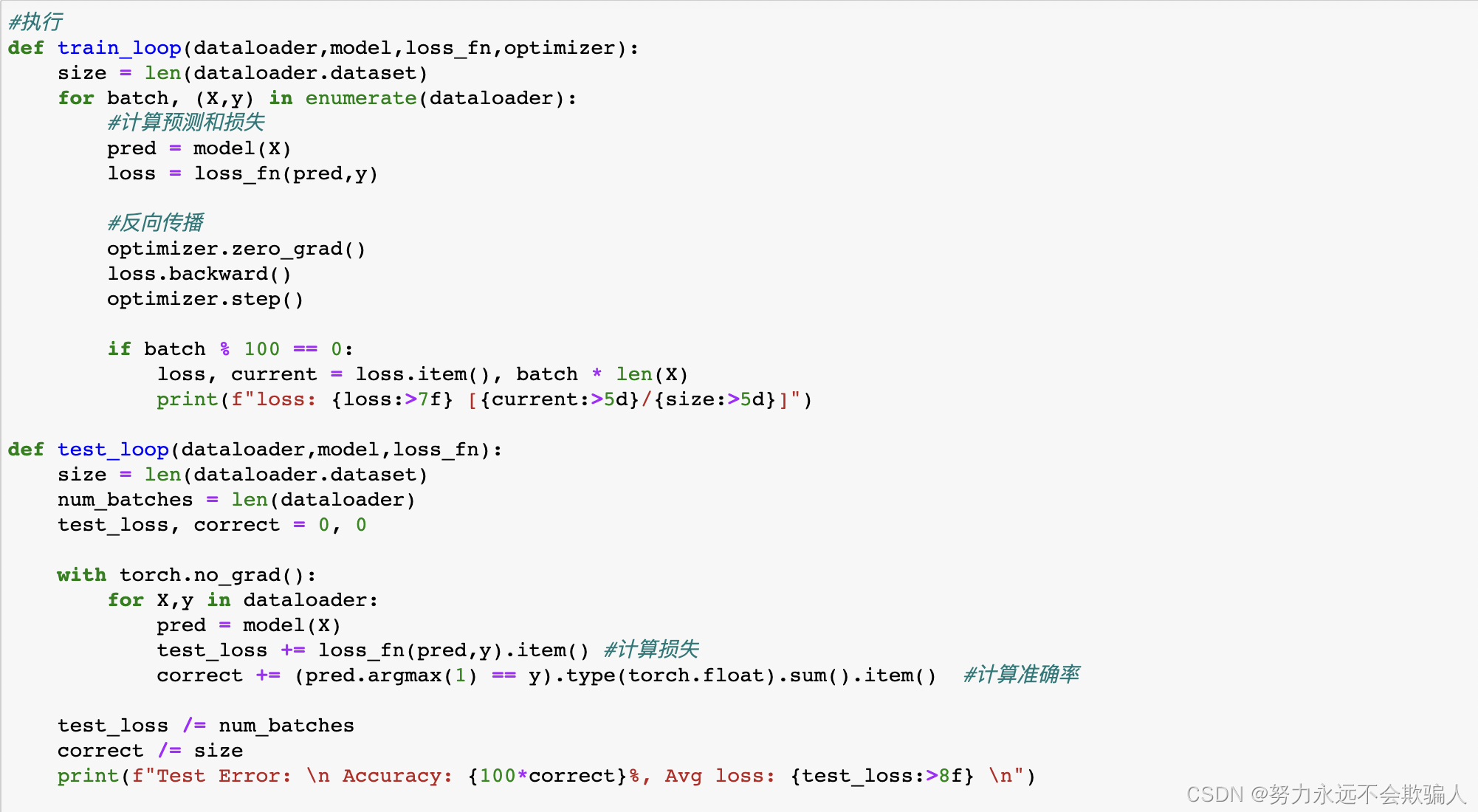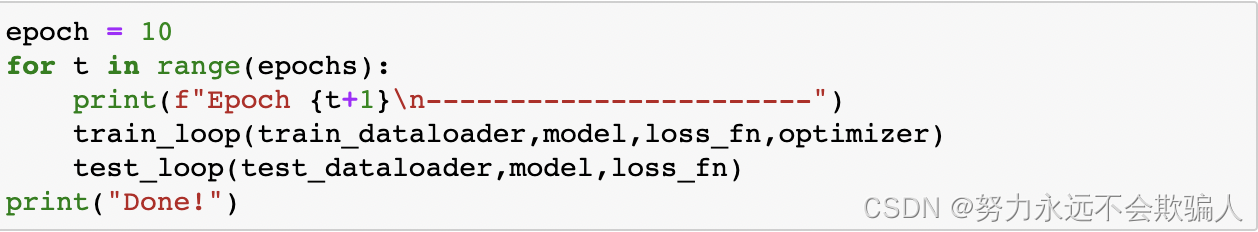```Epoch 1
-----------------------
loss: 2.168861 [    0/60000]
loss: 2.146761 [ 6400/60000]
loss: 2.093971 [12800/60000]
loss: 2.110697 [19200/60000]
loss: 2.065134 [25600/60000]
loss: 2.005016 [32000/60000]
loss: 2.029867 [38400/60000]
loss: 1.951983 [44800/60000]
loss: 1.961464 [51200/60000]
loss: 1.883326 [57600/60000]
Test Error:
Accuracy: 57.46%, Avg loss: 1.878828

Epoch 2
-----------------------
loss: 1.922104 [    0/60000]
loss: 1.871734 [ 6400/60000]
loss: 1.761671 [12800/60000]
loss: 1.804912 [19200/60000]
loss: 1.698126 [25600/60000]
loss: 1.651132 [32000/60000]
loss: 1.672112 [38400/60000]
loss: 1.571551 [44800/60000]
loss: 1.603593 [51200/60000]
loss: 1.496997 [57600/60000]
Test Error:
Accuracy: 61.82%, Avg loss: 1.507805

Epoch 3
-----------------------
loss: 1.584862 [    0/60000]
loss: 1.530016 [ 6400/60000]
loss: 1.385492 [12800/60000]
loss: 1.460753 [19200/60000]
loss: 1.349629 [25600/60000]
loss: 1.343053 [32000/60000]
loss: 1.354734 [38400/60000]
loss: 1.275000 [44800/60000]
loss: 1.315254 [51200/60000]
loss: 1.223894 [57600/60000]
Test Error:
Accuracy: 64.05%, Avg loss: 1.239179

Epoch 4
-----------------------
loss: 1.323101 [    0/60000]
loss: 1.290080 [ 6400/60000]
loss: 1.126477 [12800/60000]
loss: 1.236029 [19200/60000]
loss: 1.121515 [25600/60000]
loss: 1.143464 [32000/60000]
loss: 1.159743 [38400/60000]
loss: 1.092417 [44800/60000]
loss: 1.135134 [51200/60000]
loss: 1.064984 [57600/60000]
Test Error:
Accuracy: 64.88000000000001%, Avg loss: 1.074610

Epoch 5
-----------------------
loss: 1.149753 [    0/60000]
loss: 1.141478 [ 6400/60000]
loss: 0.959462 [12800/60000]
loss: 1.097030 [19200/60000]
loss: 0.985131 [25600/60000]
loss: 1.012667 [32000/60000]
loss: 1.040925 [38400/60000]
loss: 0.980155 [44800/60000]
loss: 1.021059 [51200/60000]
loss: 0.966461 [57600/60000]
Test Error:
Accuracy: 65.77%, Avg loss: 0.970976

Done!```

## `1、9 保存和载入模型`### `1、9、1 保存和载入模型的权重`

PyTorch模型将学习到的参数存储在内部状态字典中，称为state_dict。

2、然后加载state_dict到定义好的模型，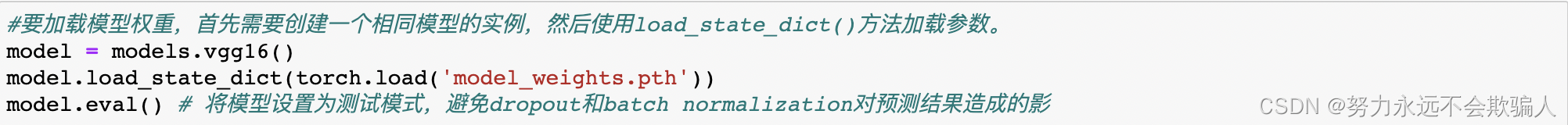```VGG(
(features): Sequential(
(0): Conv2d(3, 64, kernel_size=(3, 3), stride=(1, 1), padding=(1, 1))
(1): ReLU(inplace=True)
(2): Conv2d(64, 64, kernel_size=(3, 3), stride=(1, 1), padding=(1, 1))
(3): ReLU(inplace=True)
(4): MaxPool2d(kernel_size=2, stride=2, padding=0, dilation=1, ceil_mode=False)
(5): Conv2d(64, 128, kernel_size=(3, 3), stride=(1, 1), padding=(1, 1))
(6): ReLU(inplace=True)
(7): Conv2d(128, 128, kernel_size=(3, 3), stride=(1, 1), padding=(1, 1))
(8): ReLU(inplace=True)
(9): MaxPool2d(kernel_size=2, stride=2, padding=0, dilation=1, ceil_mode=False)
(10): Conv2d(128, 256, kernel_size=(3, 3), stride=(1, 1), padding=(1, 1))
(11): ReLU(inplace=True)
(12): Conv2d(256, 256, kernel_size=(3, 3), stride=(1, 1), padding=(1, 1))
(13): ReLU(inplace=True)
(14): Conv2d(256, 256, kernel_size=(3, 3), stride=(1, 1), padding=(1, 1))
(15): ReLU(inplace=True)
(16): MaxPool2d(kernel_size=2, stride=2, padding=0, dilation=1, ceil_mode=False)
(17): Conv2d(256, 512, kernel_size=(3, 3), stride=(1, 1), padding=(1, 1))
(18): ReLU(inplace=True)
(19): Conv2d(512, 512, kernel_size=(3, 3), stride=(1, 1), padding=(1, 1))
(20): ReLU(inplace=True)
(21): Conv2d(512, 512, kernel_size=(3, 3), stride=(1, 1), padding=(1, 1))
(22): ReLU(inplace=True)
(23): MaxPool2d(kernel_size=2, stride=2, padding=0, dilation=1, ceil_mode=False)
(24): Conv2d(512, 512, kernel_size=(3, 3), stride=(1, 1), padding=(1, 1))
(25): ReLU(inplace=True)
(26): Conv2d(512, 512, kernel_size=(3, 3), stride=(1, 1), padding=(1, 1))
(27): ReLU(inplace=True)
(28): Conv2d(512, 512, kernel_size=(3, 3), stride=(1, 1), padding=(1, 1))
(29): ReLU(inplace=True)
(30): MaxPool2d(kernel_size=2, stride=2, padding=0, dilation=1, ceil_mode=False)
)
(classifier): Sequential(
(0): Linear(in_features=25088, out_features=4096, bias=True)
(1): ReLU(inplace=True)
(2): Dropout(p=0.5, inplace=False)
(3): Linear(in_features=4096, out_features=4096, bias=True)
(4): ReLU(inplace=True)
(5): Dropout(p=0.5, inplace=False)
(6): Linear(in_features=4096, out_features=1000, bias=True)
)
)
```

### 1、9、2 保存和载入模型的形状然后我们可以像这样加载模型。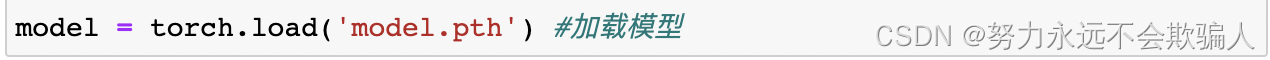### 1、9、3 将模型导出为ONNX

PyTorch也有内置的ONNX导出支持。然而，由于PyTorch执行图的动态性质，导出过程必须遍历执行图以产生持久的ONNX模型。出于这个原因，应该向导出程序传递一个适当大小的测试变量（在我们的例子中，将创建一个正确形状且值为零的tensor）。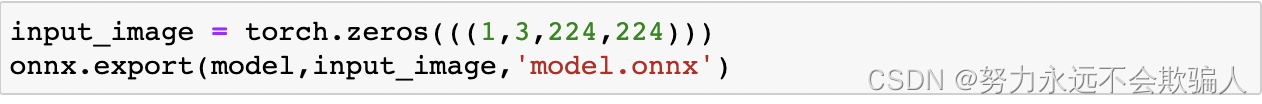你可以用ONNX模型做很多事情，包括在不同平台和不同编程语言中运行推理。关于更多细节，我们建议访问ONNX教程。### 低价透明### 金牌服务### 信息保密